# Skript

HOpenGL - 3D Graphics with Haskell
A small Tutorial
(Draft)
Sven Eric Panitz
TFH Berlin
Version Sep 24, 2004
Publish early and publish often. That is the reason why you can read this. I started playing around with HOpenGL the Haskell port of OpenGL a common library for doing 3D graphics. I more or less took minutes of my efforts and make them public in this tutorial. I did not have any prior experience in graphics programming, when I started to work with HOpenGL.
The source of this paper is an XML-file. The sources are processed by an XQuery processor, XSLT scripts and LATEX  in order to produce the different formats of the tutorial.
I'd like to thank Sven Panne1, the author of HOpenGL, who has been so kind to comment on first drafts of this tutorial.

# Contents

1  Introduction
1.1  A Little Bit of Practice
1.1.1  Opening Windows
1.1.2  Drawing into Windows
1.2  A Little Bit of Theory
1.2.2  OpenGL
1.3  A Little Bit of Technics
1.4  A Little Bit of History
2  Basics
2.1  Setting and Getting of Variables
2.1.1  Setting values
2.1.2  Getting values
2.1.3  Getting and Setting Values
2.1.4  What do the variables refer to
2.2  Basic Drawing
2.2.1  Display Functions
2.2.2  Primitive Shapes
2.2.3  Curves, Circles and so on
2.2.4  Attributes of primitives
2.2.5  Tessellation
2.2.6  Cubes, Dodecahedrons and Teapots
3  Modelling Transformations
3.1  Translate
3.2  Rotate
3.3  Scaling
3.4  Composition of Transformations
3.5.1  Shear
3.6  Some Word of Warning
3.7  Local transformations
4  Projection
4.1  The Function Reshape
4.2  Viewport: The Visible Part of Screen
4.3  Orthographic Projection
5  Changing States
5.2  Handling of Events
5.2.1  Keyboard events
5.3  Changing State over Time
5.3.1  Double buffering
5.4  Pong: A first Game
6  Third Dimension
6.1  Hidden Shapes
6.2  Perspective Projection
6.3  Setting up the Point of View
6.3.1  Oribiting around the origin
6.4  3D Game: Rubik's Cube
6.4.1  Cube Logics
6.4.2  Rendering the Cube
6.4.3  Rubik's Cube
6.5  Light
6.5.1  Defining a light source
6.5.2  Tux the Penguin

# Chapter 1 Introduction

In this chapter some basic background information can be found. You you can read the sections of this chapter in an arbitrary order. Whatever your personal preference is.

## 1.1  A Little Bit of Practice

Before you read a lot of technical details you will probably like to see something on your screen. Therefore you find some very simple examples in the beginning. This will give you a first impression, of how an OpenGL program might look like in Haskell.

### 1.1.1  Opening Windows

OpenGL's main purpose is to render some graphics on a device. This device is generally a window on your computer screen. Before you can draw something on a screen you will need to open a window. So let's have a look at the simpliest OpenGL program, which just opens an empty window:
HelloWindow
```import Graphics.UI.GLUT
import Graphics.Rendering.OpenGL

main = do
getArgsAndInitialize
createAWindow "Hello Window"
mainLoop

createAWindow windowName = do
createWindow windowName
displayCallback \$= clear [ColorBuffer]

```
The first two lines import the necessary libraries. The main function does three things:
• initialize the OpenGL system
• define a window
• start the main procedure for dispaying everything and reacting on events
For the definition of a window with a given name we do two things:
• create some window with the given name
• define, what is to be done, when the window contents is to be displayed. In the simple example above we simply clear the screen of any color by filling it with the default background color.
This 10 lines can be compiled with ghc. Do not forget to specify the packages, which contain the OpenGL library. It suffices to include the package GLUT, which automatically forces the inclusion of the package OpenGL. GLUT is the graphical user interface, which comes along with OpenGL, i.e. the window managing system etc.
```sep@swe10:~/hopengl/examples> ghc -package GLUT -o HelloWindow HelloWindow.hs
sep@swe10:~/hopengl/examples> ./HelloWindow

```
When you start the program, a window will be opened on your desktop. As you may have noticed, we did not specify any attribute of the window, like its size and position. GLUT is defined in a way that initial default values are used for unspecified attributes.

### 1.1.2  Drawing into Windows

The simple program above did just open a window. The main purpose of OpenGL is to define some graphics which is rendered in a window. Before starting to systematically explore the OpenGL library let's have a look at two examples that draw something into a window frame.

#### Some Points

First we will draw some tiny points on the screen. We use the same code for openening some window:
SomePoints
```import Graphics.UI.GLUT
import Graphics.Rendering.OpenGL

main = do
(progName,_) <- getArgsAndInitialize
createAWindow progName
mainLoop

```
The only thing that has changed, is that we make use of one of the values returned by getArgsAndInitialize: the name of the program.
For the window definition we use the code from HelloWindow.hs. But instead of clearing the screen, when the window is to be displayed, we use an own display function:
SomePoints
```createAWindow windowName = do
createWindow windowName
displayCallback \$= displayPoints

```
We want to draw some points on the screen. So let's define some points. We can do this in a list. Points in a three dimensional space are triples of coordinates. We can use floating point numbers for coordinates in OpenGL.
SomePoints
```myPoints :: [(GLfloat,GLfloat,GLfloat)]
myPoints =
[(-0.25, 0.25, 0.0)
,(0.75, 0.35, 0.0)
,(0.75, -0.15, 0.0)
,((-0.75), -0.25, 0.0)]

```
Eventually we need the display function, which displays these points.
SomePoints
```displayPoints = do
clear [ColorBuffer]
renderPrimitive Points
\$mapM_ (\(x, y, z)->vertex\$Vertex3 x y z) myPoints

```
As you see, when the window ist displayed, we want first everything to be cleared from the window. Then we use the HOpenGL function renderPrimitive. The first argument Point specifies what it is that we want to render; points in our case. For the second argument we need to transform our coordinates into some data, which is used by HOpenGL. Do not yet worry about this transformation.
As before, you will notice that again for quite a number of attributes we did not supply explicit values. We did not specify the Color of the points to be drawn. Moreover we did not define the coordinates of the graphics window. Looking at its result it is obviously a two dimensional view, where the lower left corner seems to have coordinates (-1,-1) and the upper right corner the (1,1). These values are default values chosen by the OpenGL library.

#### A Polygon

The points in the last section were rather boring? By changing a single word, we can span an area with these points. Instead of saying render the following as points, we can tell HOpenGL to render them as a polygon.
Example:
So here the program from above with one word changed. Points becomes Polygon.
APolygon
```import Graphics.UI.GLUT
import Graphics.Rendering.OpenGL

main = do
(progName,_) <-getArgsAndInitialize
createAWindow progName
mainLoop

createAWindow windowName = do
createWindow windowName
displayCallback \$= displayPoints

displayPoints = do
clear [ColorBuffer]
renderPrimitive Polygon
\$mapM_ (\(x, y, z)->vertex\$Vertex3 x y z) myPoints

myPoints :: [(GLfloat,GLfloat,GLfloat)]
myPoints =
[(-0.25, 0.25, 0.0)
,(0.75, 0.35, 0.0)
,(0.75, -0.15, 0.0)
,((-0.75), -0.25, 0.0)]

```
The resulting window can be found in figure 1.1.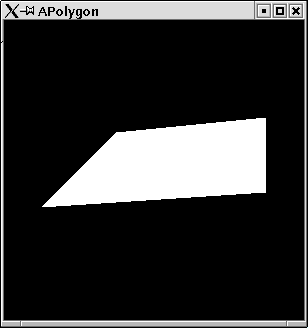Figure 1.1: A simple polygon.

## 1.2  A Little Bit of Theory

Haskell [] is a lazily evaluated functional programming language. This means that there are no mutable variables. A Haskell program consists of expressions, which do not have any side effects. Expressions are only evalutated to some value when this is absolutely necessary for program execution. This means it is hard to predict in which order subexpressions get evaluated.
Expressions evaluate to some value without changing any state. This is a nice property of Haskell, because it makes reasoning about programs easier and programs are very robust.

### 1.2.2  OpenGL

OpenGL on the other hand is a graphics library which is defined in terms of a state machine. A mutable state modells the current state of the world. Functions are executed one after another on this state in order to modify certain variables. E.g. one variable keeps the current color to which all drawing statements refer. There is a statement which allows to set the color variable to some other value.
A comprehensive introduction to OpenGL can be found in the so calledredbook[]. OpenGL comes along with a utility library called GLU [] and a system independent GUI library called GLUT [].

Having said this, Haskell and OpenGL seem to cooperate badly. There seems to be a great mismatch between the fundamental concepts of the two. However, the designers of Haskell discovered a very powerful structure, which is a perfect concept for modelling state changing functions in a purely functional language: Monads[]. Most Haskell programmers do not worry about the theory of monads but simply use them, whenever they do I/O, state changing functions or in parser construction. With monads functional programs can almost look like ordinary imperative progams [].
Monads are so essential to functional programming, that they have a special syntactic construct in Haskell, the do notation.
Print
```main = do
let x = 5
print x
let x = 6
print x
xs  <- getLine
print (length xs)

```
The monadic statements start with the keyword do. The statements have side effects. Variables can be defined and redefined in let-expressions2. Monadic statements can have a result. This can be retrieved from the statement by the <- notation.
On another aspect OpenGL and Haskell perfectly match. In OpenGL functions are assigned to different data objects, e.g. a display function is passed to windows. Since functions are first class citizens, they can easily and type safe be passed around3.

## 1.3  A Little Bit of Technics

If you want to start programming OpenGL in Haskell you need to be one of the brave, who compile sources from the functional programming CVS repository in Glasgow. There is not yet a precompiled version of the current HOpenGL library. Go to the website (www.haskell.org/ghc) of the Glasgow Haskell Compiler (GHC), follow closely the instructions on the page CVS cheat sheet. When doing the ./configure step, then use the option --enable-hopengl. i.e. start the command ./configure --enable-hopengl. This will ensure that the Haskell OpenGL library will be build and the packages OpenGL and GLUT are added to your GHC installation.
To compile Haskell OpenGL programs you simply have to add the package information to he command line invocation of GHC, i.e. use:
ghc -package GLUT MyProgram.hs
Everything else, linking etc is done by GHC. You do not have to worry about library paths or anything else.

## 1.4  A Little Bit of History

The Haskell port of OpenGL has been done by Sven Panne. Currently a stable version exists and can be downloaded as precompiled binary. This tutorial deals with the completely revised version of HopenGL, which has a more Haskell like API and needs less technical overhead. This new version is not yet available as ready to use package. You need to compile it yourself.
This tutorial has been written with no prior knowledge of OpenGL and no documentation of HOpenGL at hand.
For the old version 1.04 of HOpenGL an online tutorial written by Andre W B Furtado exists at (www.cin.ufpe.br/~haskell/hopengl/index.html) .

# Chapter 2 Basics

## 2.1  Setting and Getting of Variables

From what we have learnt in the introduction, we know that we are dealing with a state machine and will write a sequence of monadic functions which effect this machine. Before we start drawing fancy pictures let us explore the way values are set and retrieved in HOpenGL.

### 2.1.1  Setting values

The most basic operation is to assign values to variables in the state machine. In HOpenGL this is done by means of the operator \$=4 You do not need to understand, how this operator is implemented. You simply can imagine that it is an assignment operator. The left operand is a variable which gets assigned the right operand. We can revisit the first program, which simply opened a window.
Example:
When we have created a window, we assign a size to it:
Set
```import Graphics.UI.GLUT
import Graphics.Rendering.OpenGL

main = do
getArgsAndInitialize
myWindow "Hello Window"
mainLoop

myWindow name = do
createWindow name
windowSize \$= Size 800 500
displayCallback \$= clear [ColorBuffer]

```
One example of the assignment operator we have allready seen. In the last line we assign a function to the variable displayCallback. This function will be executed, whenever the window is displayed.

#### Implementation of set

The operator \$= is defined in the module
Graphics.Rendering.OpenGL.GL.StateVar as a member function of a type class:
```infixr 2 \$=

class HasSetter s where
(\$=) :: s a -> a -> IO ()

```
The variables of HOpenGL, which can be set are of type SettableStateVar e.g.:
windowTitle :: SettableStateVar String. Further variables that can be set for windows are: windowStatus, windowTitle, iconTitle, pointerPosition,

### 2.1.2  Getting values

You might want to retrieve certain values from the state. This can be done with the function get, which is in a way the corresponding function to the operator \$=.
Example:
You can retrieve the size of the screen:
Get
```import Graphics.UI.GLUT
import Graphics.Rendering.OpenGL

main = do
getArgsAndInitialize
x<-get screenSize
print x

```
When you compile and run this example the size of your screen it printed:
```sep@swe10:~/hopengl/examples> ghc -package GLUT -o Get Get.hs
sep@swe10:~/hopengl/examples> ./Get
Size 1024 768
sep@swe10:~/hopengl/examples>

```

#### Implementation of get

There is a corresponding type class, which denotes that values can be retrieved from a variable:
```class HasGetter g where
get :: g a -> IO a

```
Variables which implement this class are of type GettableStateVar a.

### 2.1.3  Getting and Setting Values

For most variables you would want to do both: setting them and retrieving their values. These variables implement both type classes and are usually of type: StateVar.
But things do not always work so simple as this sounds.
Example:
The following program sets the size of a window. Afterwards the variable windowSize is retrieved:
SetGet
```import Graphics.UI.GLUT
import Graphics.Rendering.OpenGL

main = do
getArgsAndInitialize
myWindow "Hello Window"
mainLoop

myWindow name = do
createWindow name
windowSize \$= Size 800 500
x<-get windowSize
print x
displayCallback \$= clear [ColorBuffer]

```
Running this program gives the somehow surprising result:
```sep@swe10:~/hopengl/examples> ./SetGet
Size 300 300

```
The window we created, has the expected size of (800,500) but the variable windowSize still has the default value (300,300).
The reason for this is, that setting the window size state variable has not a direct effect. It just states a wish for a window size. Only in the execution of the function mainLoop actual windows will be created by the window system. Only then the window size will be taken into account. Up to that moment the window size variable still has the default value. If you print the window size state within some function which is executed in the main loop, then you will get the actual size. By the way: you can try initialWindowSize without getting such complecated surprising results.

### 2.1.4  What do the variables refer to

The state machine contains variables and stacks of objects, which are effectedly mutated by calls to monadic functions. However not only the get and set statements modify the state but also statements like createWindow. This makes it in the beginning a bit hard to understand, when the state is changed in which way.
The createWindow statement not only constructs a window object, but keeps this new window as the current window in the state. After the createWindow statement all window effecting statements like setting the window size, are applied to this new window object.

## 2.2  Basic Drawing

### 2.2.1  Display Functions

There is a window specific variable which stores the function that is to be executed whenever a window is to be displayed, the variable displayCallback. Since Haskell is a higher order language, it is very natural to pass a function to the assignment operator. We can define a function with some arbitrary name. The function can be assigned to the variable displayCallback. In this function we can define a sequence of monadic statements.

#### Clearing the Screen

A first step we would like to do whenever the window needs to be drawn is to clear from it whatever it contains5. HOpenGL provides the function clear, which does exactly this job. It has one argument. It is a list of objects to be cleared. Generally you will clear the so called color buffer, which contains the color displayed for every pixel on the screen.
Example:
The following simple program opens a window and clears its content pane whenever it is displayed:
Clear
```import Graphics.UI.GLUT
import Graphics.Rendering.OpenGL

main = do
(progName,_) <- getArgsAndInitialize
createAWindow progName
mainLoop

createAWindow windowName = do
createWindow windowName
displayCallback \$= display

display = clear [ColorBuffer]

```

#### First Color Operations

The window in the last section has a black background. This is because we did not specify the color of the background and HOpenGL's default value for the background color is black. There is simply a variable for the background color.
For colors several data types are defined. An easy to use one is:
```data Color4 a = Color4 a a a a
deriving ( Eq, Ord, Show )

```
The four parameters of this constructor specify the red, green and blue values of the color and additionally a fourth argument, which denotes the opaqueness of the color. The values are usually specified by floating numbers of type GLfloat. Values for number attributes are between 0 and 1.
You may wonder, why there is a special type GLfloat for numbers in HOpenGL. The reason is that OpenGL is defined in a way that it is as independent from concrete types in any implementation as possible. However you do not have to worry too much about this type. You can use ordinary float literals for numbers of type GLfloat. Haskells overloading mechanism ensures that these literals can create GLfloat numbers.
Example:
This program opens a window with a red background.
BackgroundColor
```import Graphics.UI.GLUT
import Graphics.Rendering.OpenGL

main = do
getArgsAndInitialize
createAWindow "red"
mainLoop

createAWindow windowName = do
createWindow windowName
displayCallback \$= display

display = do
clearColor \$= Color4 1 0 0 1
clear [ColorBuffer]

```

#### Committing Complete Drawing

Whenever in a display function a sequence of monadic statements is defined, a final call to the function flush should be made. Only such a call will ensure that the statements are completely committed to the device, on which is drawn.

### 2.2.2  Primitive Shapes

So most preperatory things we know by now. We can start drawing onto the screen. Astonishingly in OpenGL there is only very limited number of shapes for drawing. Just points, simple lines and polygons. No curves or more complicated objects. Everything needs to be performed with these primitive drawing functions. The main function used for drawing something is renderPrimitive. The first argument of this functions specifies what kind of primitive is to be drawn. There are the following primitives defined in OpenGL:
```data PrimitiveMode =
Points
| Lines
| LineLoop
| LineStrip
| Triangles
| TriangleStrip
| TriangleFan
| Polygon
deriving ( Eq, Ord, Show )

```
The second argument defines the points which specify the primitives. These points are so called vertexes. Vertexes are actually monadic functions which constitute a point. If you want to define a point in a 3-dimensional universe with the coordinates x, y, z then you can use the following expression in HOpenGL:
vertex (Vertex3 x y z)
or, if you prefer the use of the standard prelude operator \$:
vertex\$Vertex3 x y z

#### Points

We have seen in the introductory example that we can draw points. We can simply define a vertex and use this in the function renderPrimitiv.
Example:
This program draws one single yellow point on a black screen.
SinglePoints
```import Graphics.UI.GLUT
import Graphics.Rendering.OpenGL

main = do
getArgsAndInitialize
createAWindow "points"
mainLoop

createAWindow windowName = do
createWindow windowName
displayCallback \$= display

display = do
clear [ColorBuffer]
currentColor \$= Color4 1 1 0 1
renderPrimitive Points
(vertex (Vertex3 (0.1::GLfloat) 0.5 0))
flush

```
If you do not like parantheses then you can of course use the operator \$ from the prelude and rewrite the line:
renderPrimitive Points\$vertex\$Vertex3 (0.1::GLfloat) 0.5 0
Unfortunately Haskell needs sometimes a little bit of help for overloaded type classes. Therefore you find the type annotation (0.1::GLfloat) on one of the float literals. In larger applications Haskell can usually infer this information from the context. Just in smaller applications you will sometimes need to help Haskell's type checker a bit.
The second argument of renderPrimitive is a sequence of monadic statements. So, if you want more than one point to be drawn, you can define these in a nested do statement
Example:
In this program we use a nested do statement to define more points.
MorePoints
```import Graphics.UI.GLUT
import Graphics.Rendering.OpenGL

main = do
getArgsAndInitialize
createAWindow "more points"
mainLoop

createAWindow windowName = do
createWindow windowName
displayCallback \$= display

display = do
clear [ColorBuffer]
currentColor \$= Color4 1 1 0 1
renderPrimitive Points \$
do

vertex (Vertex3 (0.1::GLfloat) 0.6 0)

vertex (Vertex3 (0.1::GLfloat) 0.1 0)
flush

```
If you want to think of points mainly as triples then you can convert a list of points into a sequence of monadic statements by first maping every triple into a vertex, e.g. by:
map (\(x,y,z)->vertex\$Vertex3 x y z)
and then combining the sequence of monadic statements into one monadic statement. Therefore you can use the standard function for monads: sequence_. The standard function mapM_ is simply the composition of map and sequence_, such that a list of triples can be converted to a monadic vertex statement by:
mapM_ (\(x,y,z) -> vertex\$Vertex3 x y z)
which is the technique used in the introductory example.
Example:
Thus we can rewrite a points example in the following way: points are defined as a list of triples. Furthermore we define some useful auxilliary functions:
EvenMorePoints
```import Graphics.UI.GLUT
import Graphics.Rendering.OpenGL

main = do
getArgsAndInitialize
createAWindow "more points"
mainLoop

createAWindow windowName = do
createWindow windowName
displayCallback \$= display

display = do
clear [ColorBuffer]
currentColor \$= Color4 1 1 0 1
let points = [(0.1,0.6,0::GLfloat)
,(0.2,0.8,0)
,(0.3,0.1,0)
,(0,0,0)
,(0.4,-0.8,0)
,(-0.2,-0.8,0)
]
renderPoints points
flush

makeVertexes = mapM_ (\(x,y,z)->vertex\$Vertex3 x y z)

renderPoints = renderAs Points

renderAs figure ps = renderPrimitive figure\$makeVertexes ps

```

#### Some useful functions

In the following we want to explore all the other different shapes which can be rendered by OpenGL. All shapes are defined in terms of vertexes which you can think of as points. We have allready seen how to define vertexes and how to open a window and such things. We provide a simple module, which will be used in the consecutive examples. Some useful functions are defined in this module.
PointsForRendering
```module PointsForRendering where
import Graphics.UI.GLUT
import Graphics.Rendering.OpenGL

```
A first function will open a window und use a given display function for the window graphics:
PointsForRendering
```renderInWindow displayFunction = do
(progName,_) <-  getArgsAndInitialize
createWindow progName
displayCallback \$= displayFunction
mainLoop

```
The next function creates for a list of points, which are expressed as triples, and a basic shape a display function which renders the desired shape.
PointsForRendering
```displayPoints points primitiveShape = do
renderAs primitiveShape points
flush

renderAs figure ps = renderPrimitive figure\$makeVertexes ps

makeVertexes = mapM_ (\(x,y,z)->vertex\$Vertex3 x y z)

```
Eventually we define a list of points as example and provide a function for easy use of these points:
PointsForRendering
```mainFor primitiveShape
= renderInWindow (displayMyPoints primitiveShape)

displayMyPoints primitiveShape = do
clear [ColorBuffer]
currentColor \$= Color4 1 1 0 1
displayPoints myPoints primitiveShape

myPoints
= [(0.2,-0.4,0::GLfloat)
,(0.46,-0.26,0)
,(0.6,0,0)
,(0.6,0.2,0)
,(0.46,0.46,0)
,(0.2,0.6,0)
,(0.0,0.6,0)
,(-0.26,0.46,0)
,(-0.4,0.2,0)
,(-0.4,0,0)
,(-0.26,-0.26,0)
,(0,-0.4,0)
]

```
Example:
We can now render the example points in a oneliner:
RenderPoints
```import PointsForRendering
import Graphics.Rendering.OpenGL

main = mainFor Points

```

#### Lines

The next basic thing to do with vertexes is to connect them, i.e.  consider them as starting and end point of a line. There are three ways to connect points with lines in OpenGL.
Singleton Lines
The most natural way is to take pairs of points and draw lines between these. This is done in the primitive mode Lines. In order that this works properly an even number of vertexes needs to be supplied to the function renderPrimitive.
Example:
Connecting our example points by lines. Pairs of points define singleton lines.
RenderLines
```import PointsForRendering
import Graphics.Rendering.OpenGL

main = mainFor Lines

```
The resulting window can be found in figure 2.1.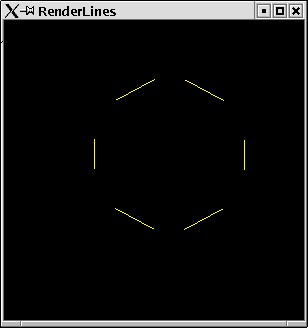Figure 2.1: Lines between points.
Line Loops
The next way to connect points with lines you probably can imagine is to make a closed figure. The end point of a line is the starting point of the next line and the last point is connected with the first, such that a closed loop of lines is created.
Example:
Now we make a loop of lines with our example points.
RenderLineLoop
```import PointsForRendering
import Graphics.Rendering.OpenGL

main = mainFor LineLoop

```
The resulting window can be found in figure 2.2.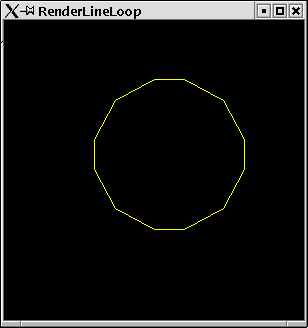Figure 2.2: A loop of lines.
Line Strip
A strip of lines is very close to a loop of lines. The only thing missing is the last line which connects the last point with the first one again.
Example:
Now we make a strip of lines with our example points.
RenderLineStrip
```import PointsForRendering
import Graphics.Rendering.OpenGL

main = mainFor LineStrip

```
The resulting window can be found in figure 2.3.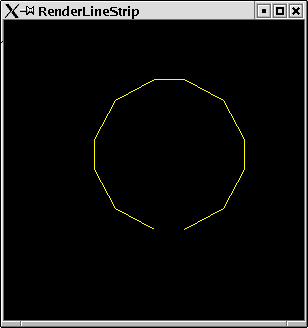Figure 2.3: A strip in terms of lines.

#### Triangles

The next basic shape which can be rendered by OpenGL are triangles. Triples of points are taken and triangles are drawn with these. As for lines there are three flavours of triangles.
Triangle
The most natural way of drawing triangles is to take triples and draw triangles. In order to work for triangles, the number of points provided needs to be a multiple of 3.
Example:
Our example vertexes define 12 points such that we get 4 triangles
RenderTriangles
```import PointsForRendering
import Graphics.Rendering.OpenGL

main = mainFor Triangles

```
The resulting window can be found in figure 2.4.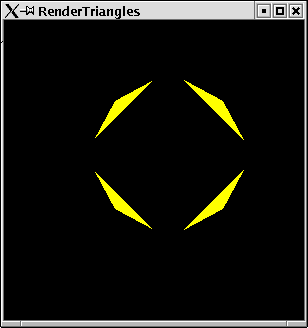Figure 2.4: Triangles.
Triangle Strips
A triangle strip makes a sequence of triangles where the next triangle uses two points of its predecessor and one new point.
Example:
For our 12 points a triangle strip will create 10 triangles.
RenderTriangleStrip
```import PointsForRendering
import Graphics.Rendering.OpenGL

main = mainFor TriangleStrip

```
The resulting window can be found in figure 2.5.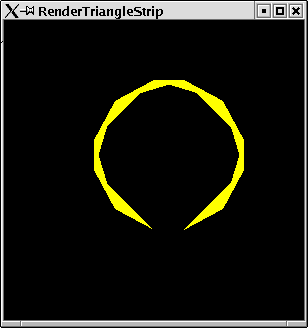Figure 2.5: A triangle strip.
TriangleFan
A fan has one starting point for all triangles. Triangles are always drawn starting from the first point.
Example:
Our example points as a fan. 10 triangles are rendered.
RenderTriangleFan
```import PointsForRendering
import Graphics.Rendering.OpenGL

main = mainFor TriangleFan

```
The resulting window can be found in figure 2.6.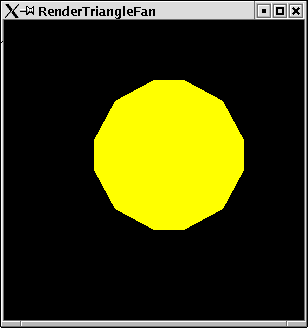Figure 2.6: A triangle strip.

Lines connected two points, triangles three points, now we will connect four points. This is calles a quad. There are two flavours of quads.
The primitive mode Quads takes quadruples of points and connects them in order to render a filled figure.
Example:
For our 12 example points OpenGL renders 3 quads
```import PointsForRendering
import Graphics.Rendering.OpenGL

```
The resulting window can be found in figure 2.7.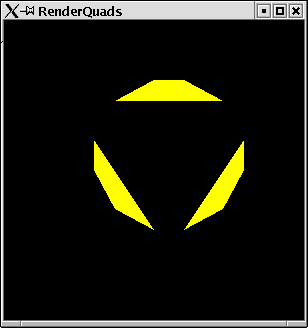In a three dimensional world quads are unlike triangles not necessarily plane areas.
For a strip of quads OpenGL uses two points of the preceeding quads for the next quad. The number n of vertexes therefore needs to be of the form: n=4+2*m.
Example:
Our examples vertexes now used for a strip of quads.
```import PointsForRendering
import Graphics.Rendering.OpenGL

```
The resulting window can be found in figure 2.8.6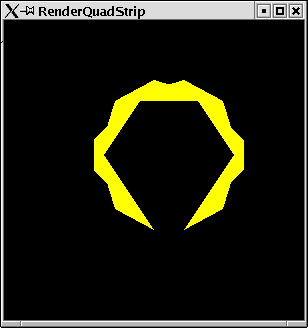#### Polygons

We connected two, three and for points. Eventually there is a shape that connects an arbitrary number of points. This is generally called a polygon. There are some restrictions for polygons:
• no convex corners are allowed.
• lines may not cross each other.
• polygons need to be planar.
Example:
Eventually our vertexes are used to define a polygon.
RenderPolygon
```import PointsForRendering
import Graphics.Rendering.OpenGL

main = mainFor Polygon

```
In this case the resulting window looks like the triangle fan we have seen before.
If you want to render polygons which hurt some of the restrictions above, you need to represent them by a set of smaller polygons. Since this is a tedious task to be done manually there is a library available, which does this for you: the GLU tessellation.

### 2.2.3  Curves, Circles and so on

In the last sections you have seen all primitive shapes, which can be rendered by OpenGL. Everything else needs to be constructed in term of these primitives. Especially you might wonder where curves and circles are. The bad news is: you have to do these by yourself.

#### Circles

With a bit mathematics you probably have allready guessed how to do curves and especially circles. You need to approximate them with a large number of lines. If the lines get very small we eventually see a curve. Let us try this with circles. We write a module which gives us some utility functions for rendering circles.
Circle
```module Circle where
import PointsForRendering
import Graphics.Rendering.OpenGL

```
The crucial function calculates a list of points which are all on the circle. You need a bit of basic geometrical knowledge for this. The coordinates of the points on a circle can be determined by sin(a) and cos(a) where a is between 0 and 2p.
Thus we can easily calculate the coordinates of an arbitrary number of points on a circle:
Circle
```circlePoints radius number
= [let alpha = twoPi * i /number
|i <- [1,2..number]]
where
twoPi = 2*pi

```
If we take a large anough number then we will eventually get a circle:
Circle
```circle radius = circlePoints radius 100

```
The following function can be used to render the circle figures:
Circle
```renderCircleApprox r n
= displayPoints (circlePoints r n) LineLoop

renderCircle r = displayPoints (circle r) LineLoop
fillCircle r = displayPoints (circle r) Polygon

```
Example:
First we test what kind of shape we get for small approximation numbers.
ApproxCircle
```import PointsForRendering
import Circle

import Graphics.Rendering.OpenGL

main = renderInWindow \$ do
clear [ColorBuffer]
renderCircleApprox 0.8 10

```
The resulting graphic can be seen in figure 2.9.Figure 2.9: 10 points on a circle.
Example:
Now we can test, if the resulting circle is, what we expected.
TestCircle
```import PointsForRendering
import Circle

import Graphics.Rendering.OpenGL

main = renderInWindow \$ do
clear [ColorBuffer]
renderCircle 0.8

```
The resulting graphic can be seen in figure 2.10.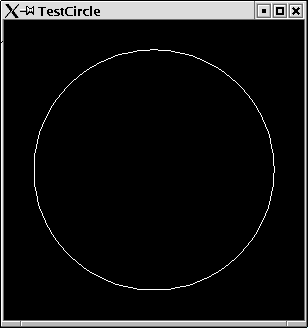Figure 2.10: Rendering a full circle.
Example:
And eventually have a look at the filled circle.
FillCircle
```import PointsForRendering
import Circle

import Graphics.Rendering.OpenGL

main
= renderInWindow \$ do
clear [ColorBuffer]
fillCircle 0.8

```
The resulting graphic can be seen in figure 2.11.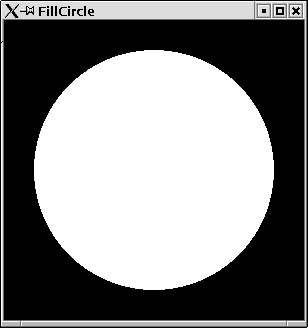Figure 2.11: A filled circle.

#### Rings

Now, where you know how to do circles, you can equally as easy define functions for rendering rings. A ring has an inner and an outer circle and fills the space between these. So we can approximate these two rings and render quads between them.
Ring
```module Ring where

import PointsForRendering
import Circle
import Graphics.Rendering.OpenGL

```
We can simply define the points of the inner and outer ring and merge these. The resulting list of points can then be rendered as a QuadStrip. Since there is no primitive mode for quad loops, we need to append the first two points as the last points again:
Ring
```ringPoints innerRadius outerRadius
= concat\$map (\(x,y)->[x,y]) (points++[p])
where
points@(p:_) = zip innerPoints outerPoints

```
Eventually we provide a small function for rendering ring shapes.
Ring
```ring innerRadius outerRadius

```
Example:
We can test the ring functions:
TestRing
```import PointsForRendering
import Ring

import Graphics.Rendering.OpenGL

main = renderInWindow \$  do
clear [ColorBuffer]
ring 0.7 0.9

```
The resulting graphic can be seen in figure 2.12.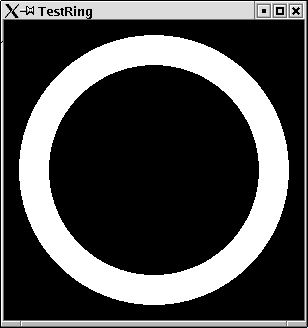Figure 2.12: A simple ring shape.

### 2.2.4  Attributes of primitives

There are some more attributes that can be set for primitive shapes (besides the color, which we have allready set).

#### Point Size

You could argue that there is no need for single points. A point can be modelled by a circle that has a small radius (or in the third dimension a sphere). However, there is something like a point in OpenGL and you can set its size. This size value for points does not refer to a radius in the coordinate system but is measured in terms of screen pixels. The default value is, one pixel per point.
Example:
We set the point size to 10 pixels:
PointSize
```import Graphics.Rendering.OpenGL
import PointsForRendering

main = renderInWindow display

display = do
pointSize \$= 10
displayMyPoints Points

```
The resulting graphic can be seen in figure 2.13.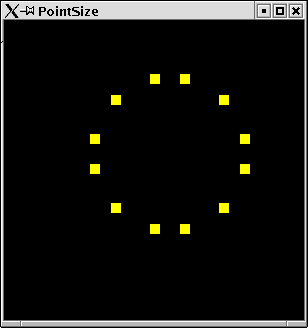Figure 2.13: Points of a large size.

#### Line Attributes

As for points, there are also further attributes for lines. First of all there is a line width. As for the point size, this is measured in screen pixels. Furthermore, you can set some line stipple: this is the pattern of the line, dashes etc. For the line stipple there is a state variable of type: Maybe (GLint, GLushort). The second argument of the value pair denotes the kind of stipple. For every short value there is one stipple. The short value has 16 bits. Every bit stands for a pixel. If for the corresponding short number the bit is set, then the pixel will be drawn, otherwise not. This means that for the short number 0 you will not see anything of your line, and for the value 65535 you will see a solid line.
The integer number of the value pair denotes a factor for the chosen stipple. For some positiv integer n every bit of the short number stands for n bits.
Example:
Setting the width of lines and a stipple:
LineAttributes
```import Graphics.Rendering.OpenGL
import Graphics.UI.GLUT  as GLUT
import PointsForRendering

main = renderInWindow display

display = do
clearColor \$= Color4 1 1 1 1
clear [ColorBuffer]
lineStipple \$= Just (1,255)
currentColor \$= Color4 0 0 0 1
lineWidth \$= 10
displayPoints squarePoints LineLoop
flush

squarePoints
= [(-0.7,-0.7,0),(0.7,-0.7,0),(0.7,0.7,0),(-0.7,0.7,0)]

```
The resulting graphic can be seen in figure 2.14.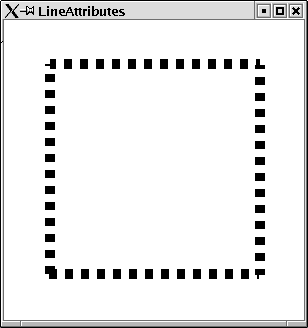Figure 2.14: Thick stippled lines.

#### Colors

You might have wondered, why the function renderPrimitive takes monadic statements as argument and not simply a list of vertexes? This means we could pass any monadic statement to the function renderPrimitive, not only statements that define vertexes by the call of the function vertex. There are some statements, which are allowed in the statements passed to renderPrimitive. One of these is setting the current color before every call of vertex to a new value. When finally rendering the primitive, OpenGL takes these color values into acount.
Example:
We define a triangle. Before the three vertexes of the triangle are defined, the current color is set to a new value.
PolyColor
```import Graphics.Rendering.OpenGL
import Graphics.UI.GLUT  as GLUT
import PointsForRendering

colorTriangle = do
currentColor \$= Color4 1 0 0 1
vertex\$Vertex3 (-0.5) (-0.5) (0::GLfloat)
currentColor \$= Color4 0 1 0 1
vertex\$Vertex3 (0.5) (-0.5) (0::GLfloat)
currentColor \$= Color4 0 0 1 1
vertex\$Vertex3 (-0.5) (0.5) (0::GLfloat)

main = renderInWindow display

display = do
clearColor \$= Color4 1 1 1 1
clear [ColorBuffer]
renderPrimitive Triangles colorTriangle
flush

```
The resulting window can be found in figure 2.15.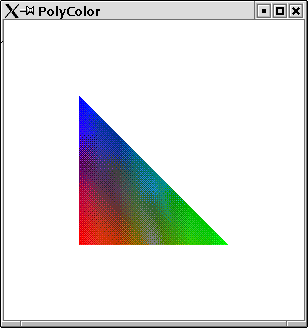Figure 2.15: A triangle with different vertex colors

### 2.2.5  Tessellation

Rendering of polygons is very limited. We cannot render polygons for crossing lines, or convex corners. Such polygons need to be expressed by a set of simpler polygons. In the module Graphics.Rendering.OpenGL.GLU.Tessellation there are a number of functions, which calculate a set of simpler polygons. For the time being, we will not go into detail, but give one single example, of how to use this library.
Example:
We want to render stars. These are shapes with convex corners.
Star
```module Star where
import Graphics.Rendering.OpenGL
import Graphics.UI.GLUT  as GLUT
import Data.Either
import Circle
import List

```
We can easily calculate the points on the star rays. They are all on one circle. We can use our function for defining circle points and get a list of points. For rendering the star, we take first the points with odd index followed by the points with even index.
Star
```starPoints radius rays
= map (\(_,(x,y,z))->Vertex3 x y z)(os++es)
where
(os,es) = partition (\(i,_)-> odd i)
\$zip [1,2..]

```
For tesselation we need to create a ComplexPolygon, which has a list of ComplexContour. A ComplexContour contains a list of AnnotatedVertexes. The annotation can be used for color or similar information. We do not make use of this annotation and simple annotate every vertex with 0.
Star
```complexPolygon points
= ComplexPolygon
[ComplexContour \$map (\v->AnnotatedVertex v 0) points]

```
The function tesselate creates a list of simple polygons. It needs some control information, which we do not explain here.
Star
```star radius rays= do
startess
<- tessellate
TessWindingPositive 0 (Normal3 0 0 0) noOpCombiner
drawSimplePolygon  startess

```
The resulting simple polygons can be rendered with the function renderPrimitive.
Star
```drawSimplePolygon  (SimplePolygon primitiveParts) =
mapM_ renderPrimitiveParts primitiveParts

renderPrimitiveParts (Primitive primitiveMode vertices) =
renderPrimitive primitiveMode
\$mapM_ (vertex . stripAnnotation) vertices

stripAnnotation (AnnotatedVertex plainVertex _) = plainVertex

noOpCombiner _newVertex _weightedProperties = 0.0 ::GLfloat

```
Now we can test our stars. We render two stars, one with 7 and one with 5 rays.
RenderStar
```import PointsForRendering
import Graphics.Rendering.OpenGL
import Graphics.UI.GLUT  as GLUT
import Star

main = renderInWindow\$do
clearColor \$= Color4 1 1 1 1
clear [ColorBuffer]

currentColor \$= Color4 1 0 0 1
star 0.9 7

currentColor \$= Color4 1 1 0 1
star 0.4 5

```
The resulting window can be found in figure 2.16.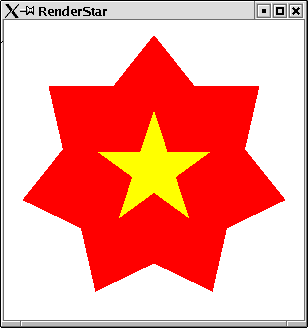Figure 2.16: RenderStar

### 2.2.6  Cubes, Dodecahedrons and Teapots

The bad news was that just very basic shapes are provided by OpenGL for rendering. The good news is that the OpenGL library comes along with a library that contains a large number of shapes.
Example:
You probably need very often the shape of a teapot. Since this is so elementary a library function is provided for this.
Tea
```import Graphics.UI.GLUT
import Graphics.Rendering.OpenGL

import PointsForRendering

main = renderInWindow display

display = do
clear [ColorBuffer]
renderObject Solid\$ Teapot 0.6
flush

```
The resulting graphic can be seen in figure 2.17.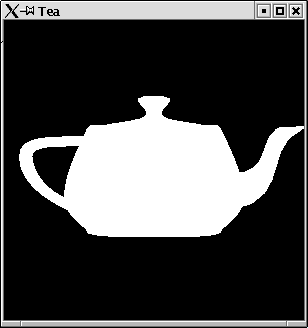Figure 2.17: A tea pot.

# Chapter 3 Modelling Transformations

By now you know, how to define different shapes for rendering. You might wonder how to place shapes on special positions or how to scale or rotate your shapes. This is done by so called transformation matrixes. Before something is rendered by OpenGL a transformation operation is performed on it. Every point will get multiplied with the transformation matrix. The transformation matrix is part of the state. So in order to transform a shape in some way, first the transformation matrix has to be set and then the shapes are to be rendered. If not specified otherwise the transformation matrix is the identity operation, i.e.  no transformation is performed. You can always reset the transformation matrix to the identity by the call of the monadic statement loadIdentity. Then the current matrix is discarded and no transformation is applied to the next rendering operations.

## 3.1  Translate

One transformation is to move a shape to another position. The according matrix is set by the statement translate. It has one argument: a vector of size three which denotes in which direction the following shapes are to be moved. Every vertex that will be rendered after a translate statement will be moved by the values of this vector.
Example:
The function ring we defined before only defined rings which have the center coordinates (0,0,0). If we want to place rings somewhere else then we need to apply a translate matrix.
SomeRings
```import PointsForRendering
import Ring
import Graphics.Rendering.OpenGL

```
We define a function, which creates a ring at a given position. Therefore we first set the transformation to the translate transformation then define the ring and finally set the transformation matrix back to the identity:
SomeRings
```ringAt x y innerRadius outerRadius = do
translate\$Vector3 x y (0::GLfloat)

```
We can test this by placing some ring in different colors on the screen.
SomeRings
```main = do
renderInWindow  someRings

someRings = do
clearColor \$= Color4 1 1 1 1
clear [ColorBuffer]

currentColor \$= Color4 1 0 0 1
ringAt 0.5 0.3 0.1 0.12

currentColor \$= Color4 0 1 0 1
ringAt (-0.5) 0.3 0.3 0.5

currentColor \$= Color4 0 0 1 1
ringAt (-1) (-1) 0.7 0.75

currentColor \$= Color4 0 1 1 1
ringAt 0.7 0.7 0.2 0.3

```
The resulting graphic can be seen in figure 3.1.
Note that if we did not reset the transformation back to the identity, we would get the composition of all transformations.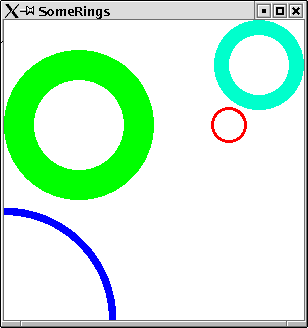Figure 3.1: Rings translated to different positions.

## 3.2  Rotate

Another transformation that can be performed is rotation. The rotate statement has two arguments. The first one specifies by which degree the following shapes are to be rotated counterclockwise. The second argument is a vector which specifies around which axis the shape is to be rotated.
Example:
In this example we apply the composition of two transformations. Squares are moved to some position and furthermore rotated around the z-axis.
We write a simple module for rendering filled rectangles:
Squares
```module Squares where

import Graphics.Rendering.OpenGL
import PointsForRendering

```
Here is a function for arbitrary rectangles:
Squares
```myRect width height =
where
w = width/2
h = height/2

```
A square is just a special case:
Squares
```square width = myRect width width

```
Now we will transform squares.
SomeSquares
```import PointsForRendering
import Squares
import Graphics.Rendering.OpenGL

```
We define a function, which applies the rotate transformation to a square. It is rotated around the z-axis.
SomeSquares
```rotatedSquare alpha width  = do
rotate alpha \$Vector3 0 0 (1::GLfloat)
square width

```
A further utility function moves some shape to a specified position. Note that this function resets the matrix again.
SomeSquares
```displayAt x y displayMe = do
translate\$Vector3 x y (0::GLfloat)
displayMe

```
Some squares are defined and rotated:
SomeSquares
```main = do
renderInWindow  someSquares

someSquares = do
clearColor \$= Color4 1 1 1 1
clear [ColorBuffer]

currentColor \$= Color4 1 0 0 1
displayAt 0.5 0.3\$rotatedSquare  15 0.12

currentColor \$= Color4 0 1 0 1
displayAt (-0.5) 0.3\$rotatedSquare 25 0.5

currentColor \$= Color4 0 0 1 1
displayAt (-1) (-1)\$rotatedSquare 4 0.75

currentColor \$= Color4 0 1 1 1
displayAt 0.7 0.7\$rotatedSquare 40 0.3

```
The resulting graphic can be seen in figure 3.2.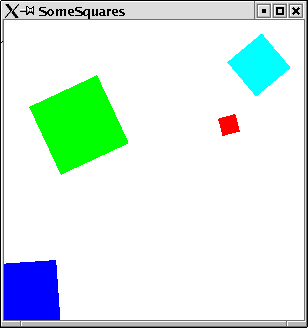Figure 3.2: Squares translated and rotated.

## 3.3  Scaling

The third transformation enables you to scale shapes. This is not only useful for changing the size of some object but for stretching it in some direction. The transformation scale has three arguments, which represent the scaling factors in the three dimensional space.
Example:
We apply three transformations on the tea pot example. We rotate and translate it and finally we stretch it a bit by a scale transformation.
Coffee
```import Graphics.UI.GLUT
import Graphics.Rendering.OpenGL

import PointsForRendering
main = renderInWindow display

display = do
clear [ColorBuffer]
scale 0.3 0.9 (0.3::GLfloat)
translate\$Vector3 (-0.3) 0.3 (0::GLfloat)
rotate 30 \$Vector3 0 1 (0::GLfloat)
renderObject Solid\$ Teapot 0.6
flush

```
The resulting graphic can be seen in figure 3.3. As you see it looks now like a coffee pot.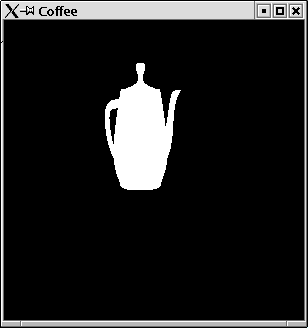Figure 3.3: A coffee pot.
Remember that the scale and the rotate transformation always refer to the origin (0,0,0) of your coordinates. Rotating an object, which is not situated at the origin will move it around the origin. Scaling an object which is not situated at the origin might deform the object in surprising ways.

## 3.4  Composition of Transformations

Since Haskell is a functional programming language let us think of transformations as functions. A transformation is a function that is applied to every vertex before it is rendered. If you define two transformations for an object, e.g. a rotation and a translation, then you define a composition of these transformations.
The code:
```rotatedSquareAt width alpha x y z = do
translate\$Vector3 x w y
rotate alpha \$Vector3 0 0 (1::GLfloat)
square width

```
defines a composition of a translate und a rotate transformation, which is applied to a square figure. A sequence of transformation statements is composed to a single transformation in the same way as the standard function composition operator (.) composes functions: (f . g) x = f(g(x)). The compositional function (f . g) is the same as first applying function g and then applying f. For transformations in HOpenGL this means that for a sequence of transformations
```translate\$Vector3 x w y
rotate alpha \$Vector3 0 0 (1::GLfloat)

```
first the points are rotated and then they are translated.
The order in which transformations are performed is of course not arbitrary. A rotation after a translation is different to a translation after a rotation.
Example:
This example illustrates the different compositions of rotation and translation.
Compose
```import PointsForRendering
import Squares
import Graphics.Rendering.OpenGL

displayAt x y displayMe = do
displayMe

main = do
renderInWindow  someSquares

someSquares = do
clearColor \$= Color4 1 1 1 1
clear [ColorBuffer]

```
A black square at the origin:
Compose
```  currentColor \$= Color4 0 0 0 1
square 0.5

```
A blue square translated:
Compose
```  currentColor \$= Color4 0 0 1 1
translate\$Vector3 0.5 0.5 (0::GLfloat)
square 0.5

```
A light blue square that is rotated:
Compose
```  currentColor \$= Color4 0 1 1 1
rotate 35 \$Vector3 0 0 (1::GLfloat)
square 0.5

```
A red square that is first rotated and then translated:
Compose
```  currentColor \$= Color4 1 0 0 1
translate\$Vector3 0.5 0.5 (0::GLfloat)
rotate 35 \$Vector3 0 0 (1::GLfloat)
square 0.5

```
A yellow square that is first translated and then rotated:
Compose
```  currentColor \$= Color4 1 1 0 1
rotate 35 \$Vector3 0 0 (1::GLfloat)
translate\$Vector3 0.5 0.5 (0::GLfloat)
square 0.5

```
The resulting window can be found in figure 3.4.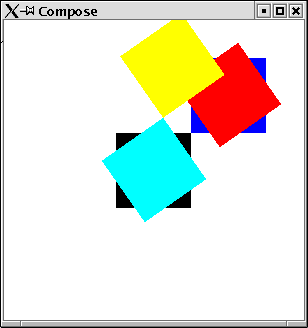Figure 3.4: Different compositions of translation and rotation.
Since the scale und the rotate transformation refer both to the origin and the translate transformation can move objects away from the origin it is a good policy to create objects at the origin, then rotate and scale it and finally translate it to its final position. Therefore predefined shapes in the library are usually positioned at the origin, as e.g. the tea pot.

## 3.5  Defining your own transformation

The three ready to usee transformations rotation, scaling and translation or their composition might not suffice for your needs. Then you can define your own transformations. Technically a transformation in OpenGL is represented as a matrix. Every vertex gets multiplied by the transformation matrix before it is rendered. In order to define a transformation, we will need to construct such a matrix.
Internally every vertex in OpenGL is not represented by 3 coordinates (x,y,z) but by four coordinates (x,y,z,w). The x, y, z values are devided by w. Usually the value of w is 1.0.
Thus for a transformation matrix you need a matrix of four rows and four columns. Remember that a matrix is multiplied with a vector in the following way:

 x11
 x12
 x13
 x14
 x21
 x22
 x23
 x24
 x31
 x32
 x33
 x34
 x41
 x42
 x43
 x44

 x
 y
 z
 w

=

 x11 * x + x12 * y + x13 * z + x14 * w
 x21 * x + x22 * y + x23 * z + x24 * w
 x31 * x + x32 * y + x33 * z + x34 * w
 x41 * x + x42 * y + x43 * z + x44 * w

OpenGL provides a function for creation of a transformation matrix out of a list: matrix. It takes as first argument a parameter, which specifies in which order the matrix elements appear in the list: RowMajor for row wise and ColumMajor for column wise appearance. The function multMatrix allows to multiply your newly created transformation matrix to the current transformation context.

### 3.5.1  Shear

We can now define our own transformations. We can define the transformation shear. Mathematical textbooks define shear in the following way:
A transformation in which all points along a given line L remain fixed while other points are shifted parallel to L by a distance proportional to their perpendicular distance from L. Shearing a plane figure does not change its area.
Eric Weissteins's world of mathematics (http://mathworld.wolfram.com/Shear.html)
We define a shear transformation, which leaves y and z coordinates unchanged, and adds to the x coordinate some value depending on the value of y. For some f we need the following transformation matrix:

 1
 f
 0
 0
 0
 1
 0
 0
 0
 0
 1
 0
 0
 0
 0
 1

 x
 y
 z
 w

=

 x + f * y
 y
 z
 w

As you can see, this is almost the identity. We can define this in HOpenGL:
MyTransformations
```module MyTransformations where
import Graphics.Rendering.OpenGL
import Graphics.UI.GLUT  as GLUT

shear f = do
m <-  (newMatrix RowMajor [1,f,0,0
,0,1,0,0
,0,0,1,0
,0,0,0,1])
multMatrix (m:: GLmatrix GLfloat)

```
Let us test our new transformation:
TestShear
```import PointsForRendering
import Circle
import Squares
import MyTransformations

import Graphics.Rendering.OpenGL
import Graphics.UI.GLUT  as GLUT

main = renderInWindow\$do
clearColor \$= Color4 1 1 1 1
clear [ColorBuffer]
translate\$Vector3 0.5 0.5 (0::GLfloat)
shear 0.5
currentColor \$= Color4 0 0 1 1
fillCircle 0.5

translate\$Vector3 (-0.5) (-0.5) (0::GLfloat)
shear 0.5
currentColor \$= Color4 1 0 0 1
square 0.5

```
The resulting window can be found in figure 3.5.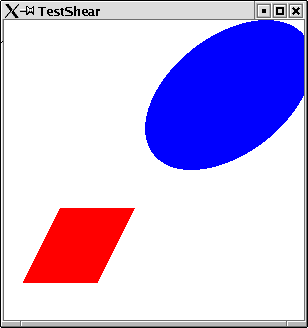Figure 3.5: Applying shear to some shapes.

## 3.6  Some Word of Warning

You might get strange effects when you forget to reset the transformation matrix. This might not only effect further rendering statements but also applies to the redisplay of your window. The display function you specified for your window will be called whenever the window needs to be displayed. However this does not automatically reset the transformation matrix to the identity matrix. This results in the effect that every redisplay of your window changes its contents.
Example:
In this example a ring is displayed. Each time the display function is called the contents of the ring moves a bit. Compile the program and hide the resulting window behind some other window. You will observe how the ring moves within the window, until it is no longer displayed.
ForgottenReset
```import Graphics.UI.GLUT
import Graphics.Rendering.OpenGL

import PointsForRendering
import Ring
import Squares

main = renderInWindow display

display = do
clear [ColorBuffer]
translate\$Vector3 (-0.1) 0.1 (0::GLfloat)
ring 0.2 0.4
flush

```
As a matter of fact this effect may not only occur with transformations, but every state changing statement. If you set the color as last statement in your display function to some value then this will be the current color in the next call of the display function. Thus it is better to ensure that the display function leaves a clean state, i.e. the state it espects to find, when it is called, or even better let the display functions not rely on any previously set states.

## 3.7  Local transformations

Often you will have the situation, that you are in a context of some transformations. Maybe for certain parts of you shape you want to add some further transformation but for other parts return to the outer transformation context. In such situations you cannot use the statement loadIdentity since this will not only delete the transformations you wanted to be applied to your local part of the the complete shape but the whole transformation context.
HOpenGL provides a function which allows to add some more transformations to some local parts of your shape. This function is called preservingMatrixs which refers to the fact that transformations are technically implemented as matrixes. preservingMatrix has one argument, which is a monadic statement. The application of preservingMatrix is a monadic statement:
```preservingMatrix :: IO a -> IO a

```
Every transformation done within this monadic statement will not be done only locally. It does not effect the statements which follow after the application of preservingMatrix.
Example:
To demonstrate the use of preservingMatrix we provide a module, which is able to render a side of the famous Rubik's Cube. Such a side consists of 9 squares which are of some color and which have a black frame. We can render such a shape, by rendering the single framed squares at the origin and then move them to their position. This movement is done within a preservingMatrix application.
RubikFace
```module RubikFace where
import Graphics.UI.GLUT
import Graphics.Rendering.OpenGL

import Squares
import PointsForRendering

```
Doing a frame involves the four sides of a frame. Each side is created at the origin and then moved to its final position:
RubikFace
```frame width height border = do
let bh = border/2
let wh = width/2-bh
let hh = height/2-bh

preservingMatrix \$ do
translate \$Vector3 0 hh (0::GLfloat)
myRect width  border
preservingMatrix \$ do
translate \$Vector3 0 (-hh) (0::GLfloat)
myRect width  border
preservingMatrix \$ do
translate \$Vector3 (-wh) 0 (0::GLfloat)
myRect  border height
preservingMatrix \$ do
translate \$Vector3 wh 0 (0::GLfloat)
myRect  border height

```
Each of the nine fields is rendered by drawing its frame and its colored square:
RubikFace
```originField width color = do
let frameWidth = width/10
currentColor \$= Color4 0 0 0 1
frame width width frameWidth
let sc = 18/20::GLfloat
currentColor \$= color
square (width-frameWidth)

```
Eventually the side of Rubik's Cube can be drawn
RubikFace
```renderArea :: GLfloat -> [[Color4 GLfloat]] -> IO ()
renderArea width css
= do
let cs  = concat css
cps = zip cs \$ areaFields width
mapM_  (\(c,f)-> f(originField width c)) cps

areaFields width =
[makeSquare x y |x<-[1,0,-1],y<-[1,0,-1]]
where
makeSquare xn yn = \f -> preservingMatrix \$ do
let
x = xn*width
y = yn*width
translate \$Vector3 x y 0
f

red     = Color4 1 0 0 (1::GLfloat)
green   = Color4 0 1 0 (1::GLfloat)
blue    = Color4 0 0 1 (1::GLfloat)
yellow  = Color4 1 1 0 (1::GLfloat)
white   = Color4 1 1 1 (1::GLfloat)
black   = Color4 0 0 0 (1::GLfloat)

```
The following module tests the rendering. Two sides are rendered. Further transformations are applied to them.
RenderRubikFace
```import PointsForRendering
import Graphics.Rendering.OpenGL

import PointsForRendering
import RubikFace

_FIELD_WIDTH :: GLfloat
_FIELD_WIDTH = 1/5

main =  renderInWindow  faces

faces = do
clearColor \$= white
clear [ColorBuffer]

translate  \$Vector3 (-0.6) 0.4 (0::GLfloat)
renderArea _FIELD_WIDTH r1

translate  \$Vector3 (0.1) (-0.3) (0::GLfloat)
rotate 290 \$ Vector3 0 0 (1::GLfloat)
scale 1.5 1.5 (1::GLfloat)
renderArea _FIELD_WIDTH r1

r1=[[red,blue,yellow],[white,green,red],[green,yellow,blue]]

```
The resulting window can be found in figure 3.6.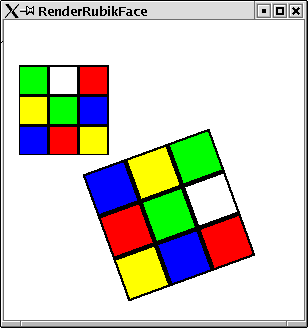Figure 3.6: a Side of Rubik's Cube with further transformations applied to it.

# Chapter 4 Projection

## 4.1  The Function Reshape

Up to now we always relied on the default values for most attributes which are concerned with projection. From where do we look at the scenery? Which coordinates are displayed to what extend on the screen. Such attributes can be set in the reshape callback function. This function gets the window size as argument and specifies which coordinates are to be seen on the screen. At first glance the name seems to be a bit misleading, since it evokes the image that it is just called, when someone resizes the window. The first time the reshape function is called is at the opening of the window.
The reshape function might be empty. This is modelled by the Haskell data type Maybe.
Example:
We define the first reshape function for a window. It is the identity function, which does not specify anything, how to render the picture.
Reshape1
```import Graphics.UI.GLUT
import Graphics.Rendering.OpenGL

import PointsForRendering

main = do
(progName,_) <- getArgsAndInitialize
createWindow progName
displayCallback \$= display
reshapeCallback \$= Just reshape
mainLoop

display = do
clear [ColorBuffer]
where
points
= [(0.5,0.5,0)
,(-0.5,0.5,0)
,(-0.5,-0.5,0)
,(0.5,-0.5,0)]

reshape s = return ()

```
Run this example. You will see a white square in the middle of a black screen. Now resize the window. You will notice that the size of the square will not change. If you make the window smaller parts of the picture are not displayed, if you enlarge the window parts of the window contain no image (which means it might be some arbitrary image). Figure  4.1 shows how the window looks after enlarging it a bit.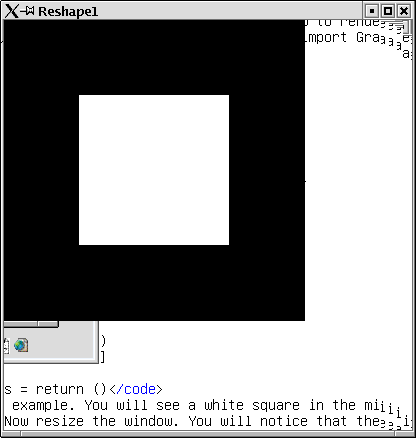Figure 4.1: Enlarging a window with the empty reshape function.

## 4.2  Viewport: The Visible Part of Screen

Usually you want to define in the reshape function, which parts of the window pane are to be used for rendering the picture. There is a state variable viewport, which contains exactly this information. It is a pair, of a position and a size. The position is the offset from the upper left corner in pixels. The size is the size of the screen to be used for rendering in pixels.
Example:
If you want the window to be used completely for rendering the image, then the position needs to be set to Position 0 0. i.e. no offset and as size the complete window size is to be used:
Reshape2
```import Graphics.UI.GLUT
import Graphics.Rendering.OpenGL

import PointsForRendering

main = do
(progName,_) <- getArgsAndInitialize
createWindow progName
displayCallback \$= display
reshapeCallback \$= Just reshape
mainLoop

display = do
clear [ColorBuffer]
where
points
= [(0.5,0.5,0)
,(-0.5,0.5,0)
,(-0.5,-0.5,0)
,(0.5,-0.5,0)]

reshape s@(Size w h) = do
viewport \$= (Position 0 0, s)

```
If you start this program and resize the window, then always the complete window pane will be used for rendering your image.
Example:
In this example only parts of the window are used for rendering the image. The image is smaller than the window.
Viewport
```import Graphics.UI.GLUT
import Graphics.Rendering.OpenGL

import PointsForRendering

main = do
(progName,_) <- getArgsAndInitialize
createWindow progName
clearColor \$= Color4 0 0 0 0
displayCallback \$= display
reshapeCallback \$= Just reshape
mainLoop

display = do
clearColor \$= Color4 1 1 1 1
clear [ColorBuffer]
currentColor \$= Color4 1 0 0 1
displayPoints ps1 LineLoop
displayPoints ps2 Lines
where
ps1=[(0.5,0.5,0),(-0.5,0.5,0),(-0.5,-0.5,0),(0.5,-0.5,0)]
ps2=[(1,1,0),(-1,-1,0),(-1,1,0),(1,-1,0) ]

reshape s@(Size w h) = do
viewport \$= (Position 50 50, Size (w-80) (h-60))

```
The resulting window can be found in figure 4.2.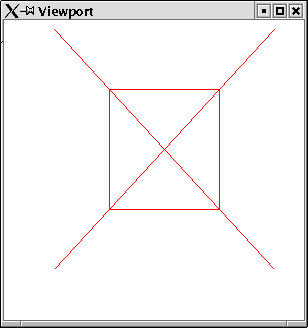Figure 4.2: A Viewport smaller than the window.

## 4.3  Orthographic Projection

The viewport defines which parts of your window pane are used for rendering your image. The actual projection defines which coordinates you want to display. The simpliest way to specify this is by the function ortho. It has six arguments, the lower and upper bounds of the x, y, z coordinates.
Projection is equally as transformation internally expressed in terms of a matrix. The statement loadIdentity can refer to the transformation or to the projection matrix. A state variabble matrixMode defines, which of these matrixes these statements refer to. Therefore it is necessary to switch this variable to the value Projection, before applying the function ortho and afterwards to reset the variable back to the value ModelView.
Example:
We render the same image in two windows with different projection values:
Ortho
```import PointsForRendering
import Graphics.Rendering.OpenGL
import Graphics.UI.GLUT  as GLUT
import Star

main = do
(progName,_) <-  getArgsAndInitialize

createWindow (progName++"1")
displayCallback \$= display
projection (-5) 5 (-5) 5 (-5) 5

createWindow (progName++"2")
displayCallback \$= display
projection 0 0.8 (-0.8) 0.8 (-0.5) 0.5

mainLoop

projection xl xu yl yu zl zu = do
matrixMode \$= Projection
ortho xl xu yl yu zl zu
matrixMode \$= Modelview 0

display = do
clearColor \$= Color4 1 1 1 1
clear [ColorBuffer]
currentColor \$= Color4 1 0 0 1
star 0.9 7
currentColor \$= Color4 1 1 0 1
star 0.4 5

```
The resulting windows can be found in figure 4.3.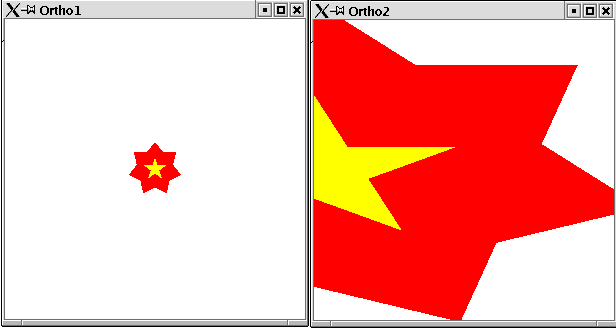Figure 4.3: Two windows with different projection.
ortho is the simpliest projection we can define. When we will consider third dimensional szeneries we will learn a more powerful projection.

# Chapter 5 Changing States

OpenGL is not only designed to render static images, but to have changing images. There are to ways how your image might change:
• it might react to some event, like some keyboard input or mouse event.
• it might change over time.
In order to change your image in some coordinated way, you need a state which can change. An event may change your state, or over the time your state might be changed.

## 5.1  Modelling your own State

A state is of course something, which does not match the purely functional paradigm of Haskell. However in the context of I/O the designers of Haskell came up with some clever way to integrate state changing variables into the Haskell's purely functional setting. The trick are again monads, as you have seen before for the state machine of OpenGL. There is a standard library in Haskell for state changing variables: Data.IORef. This provides functions for creation, setting, retrieving and modification of state variables. These functions are called:
If you think these names a bit too technical, then you might use the following module, which makes IORef variables instances of the type classes HasGetter and HasSetter. Thus we can use our own state variables in the same way, we use the HOpenGL state variables.7
StateUtil
```module StateUtil where

import Graphics.Rendering.OpenGL
import Data.IORef
import Graphics.UI.GLUT

--instance HasSetter IORef  where
--  (\$=) var val = writeIORef var val

--instance HasGetter IORef  where
--  get var = readIORef var

new = newIORef

```

## 5.2  Handling of Events

Now we know how to modell our own state. We can use this for reacting on some events. Event handling in HOpenGL is done by setting a callback function for mouse and keyboard events. A callback function for mouse and keyboard events needs to be of the following type:
```type KeyboardMouseCallback =
Key -> KeyState -> Modifiers -> Position -> IO ()

```
A Key can be some character, some special character or some mouse buttom:
```data Key
= Char Char
| SpecialKey SpecialKey
| MouseButton MouseButton
deriving ( Eq, Ord, Show )

```
The keystate informs, if the key has been pressed or released.
```data KeyState
= Down
| Up
deriving ( Eq, Ord, Show )

```
A modifier denotes, if some extra key is used, like the alt, strg or shift key:
```data Modifiers = Modifiers { shift, ctrl, alt :: KeyState }
deriving ( Eq, Ord, Show )

```
And finally the position informs about the current mouse pointer position.

### 5.2.1  Keyboard events

With the close look at the event handling function above it is fairly easy to write a program that reacts on keyboard events. A function of type KeyboardMouseCallback is to be written and assigned to the state variable keyboardMouseCallback of your window. Usually your KeyboardMouseCallback will have access to some of your state variables, since you want to change a state when an event occurs. When the state has been changed, HOpenGL needs to be forced to redisplay the picture with the new state values. Therefore a call to the function postRedisplay needs to be done.
Example:
In this example we draw a circle. The radius of the circle can be changed by use of the + and - key.
State
```import Circle
import PointsForRendering
import StateUtil

import Graphics.Rendering.OpenGL
import Data.IORef
import Graphics.UI.GLUT

main = do
(progName,_) <-  getArgsAndInitialize
createWindow progName

```
We create a state variable which stores the current radius of the circle:
State
```  radius <- new 0.1

```
The display function gets this state variable as first argument:
State
```  displayCallback \$= display radius

```
And the keyboard callback gets this variable as first argument:
State
```  keyboardMouseCallback \$= Just (keyboard radius)
mainLoop

```
The display function gets the current value for the radius and draws a filled circle:
State
```display radius = do
clear [ColorBuffer]
fillCircle r

```
The keyboard callback reacts on two keyboard events. The value of the radius variable are changed:
State
```keyboard radius (Char '+') Down _ _ = do
postRedisplay Nothing
keyboard radius (Char '-') Down _ _ = do
postRedisplay Nothing
keyboard _ _ _ _ _ = return ()

```
Compile and start this program and press the + and - key.

## 5.3  Changing State over Time

The second way to change your picture is over time. You can create an animation if your picture changes a tiny bit every moment. In HOpenGL you can a define a so called idle function. This function will be evaluated whenever the picture has been displayed. There you can define, in what way your state will change before the next redisplay is performed. The last statement in an idle function will be usually a call to postRedisplay.
Example:
We define our first animation. A ring is displayed with a changing radius.
Idle
```import Ring
import PointsForRendering
import StateUtil

import Graphics.Rendering.OpenGL
import Data.IORef
import Graphics.UI.GLUT  as GLUT

```
We define a constant which denotes the value by which the radius changes between every redisplay:
Idle
```_STEP = 0.001

```
Within the main function an idle callback is added to the window:
Idle
```main = do
(progName,_) <-  getArgsAndInitialize
createWindow progName
step   <- new _STEP
idleCallback \$= Just (idle radius step)
mainLoop

```
The display function renders a ring, depending on the state variable for the radius:
Idle
```display radius = do
clear [ColorBuffer]
ring r (r+0.2)
flush

```
The idle function changes the value of the variable radius depending on the second state variable step.
Idle
```idle radius step = do
s <- get step
if r>=1 then step \$= (-_STEP)
else if r<=0 then step \$= _STEP
else return ()
s <- get step
postRedisplay Nothing

```

### 5.3.1  Double buffering

The animation created in the last example was not very satisfactory. A ring with changing radius was displayed, but the animation was somehow flickering. The reason for that was, that the display function as its first statement clears the screen, i.e. makes it alltogether black. Only afterwards the ring is rendered. For a short moment the screen will be completely black. This is what makes this flickering effect.
A common solution for this problem in animated pictures is, not to apply the statements of the display function directly to the screen, but to an invisible buffer. When all statements of the display function have been applied to this invisible background buffer, this buffer is copied to the screen. This way only the ready to use final picture is shown on screen and not any intermediate rendering step (e.g. the picture after the clear statement).
OpenGL provides a double buffering mechanism. We only have to activate this. Therefore we need to set the initial display mode variable accordingly. Instead of a call to the function flush a call to the function swapBuffers needs to be done as last statement of the display function.
Example:
The ring with changing radius over time now with double buffering.
Double
```import Ring
import PointsForRendering
import StateUtil

import Graphics.Rendering.OpenGL
import Data.IORef
import Graphics.UI.GLUT  as GLUT

_STEP = 0.001

main = do
(progName,_) <-  getArgsAndInitialize
initialDisplayMode \$= [DoubleBuffered]
createWindow progName
step   <- new _STEP
idleCallback \$= Just (idle radius step)
mainLoop

clear [ColorBuffer]
ring r (r+0.2)
swapBuffers

s <- get step
if r>=1 then step \$= (-_STEP)
else if r<=0 then step \$= _STEP
else return ()
s <- get step
postRedisplay Nothing

```

## 5.4  Pong: A first Game

By now you have seen a lot of tiny examples. It is time to draw the techniques together and do an application with HOpenGL. In this section we will implement one of the first animated computer games ever: Pong. It consists of a small white circle which moves over a black screen and two paddles which can move on a vertical line.
Pong in action can be found in figure 5.1.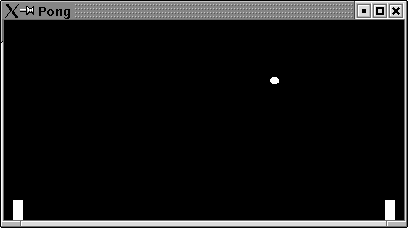Figure 5.1: Pong in action.
Pong
```import Circle
import Squares
import PointsForRendering
import StateUtil

import Graphics.Rendering.OpenGL
import Data.IORef
import Graphics.UI.GLUT  as GLUT

```
First of all we define some constant values for the game: x-, y-coordinates of the game, width and height of a paddle, the radius of the ball, initial factor, how a ball and a paddle changes its position, and an initial board size.
Pong
```_LEFT  = -2
_RIGHT =  1
_TOP   =  1
_BOTTOM= -1

_INITIAL_WIDTH :: GLsizei
_INITIAL_WIDTH=400

_INITIAL_HEIGHT::GLsizei
_INITIAL_HEIGHT=200

_INITIAL_BALL_DIR = 0.002

```
We define a data type, game. The game state can be characterized by the position of the ball and the values these coordinates change for the next redisplay:
Pong
```data Ball   = Ball (GLfloat,GLfloat) GLfloat GLfloat

```
The paddles, which are characterized by their position and the position change on the y-axis (x-axis is fixed for a paddle).
Pong
```type Paddle = (GLfloat,GLfloat,GLfloat)

```
Additionally a game has points for the left and the right player and a factor which denotes how fast ball and paddles move:
Pong
```data Game
= Game { ball ::Ball
, points ::(Int,Int)
, moveFactor::GLfloat}

```
For a starting game we provide the following initial game state:
Pong
```initGame
= Game {ball=Ball (-0.8,0.3) _INITIAL_BALL_DIR _INITIAL_BALL_DIR
,points=(0,0)
,moveFactor=1
}

```
The main function creates a double buffering window in fullscreen mode. An initial game state is created and passed to the keyboard, display, idle and reshape function:
Pong
```main = do
(progName,_) <-  getArgsAndInitialize
initialDisplayMode \$= [DoubleBuffered]
createWindow progName
game <- newIORef initGame
--windowSize \$= Size _INITIAL_WIDTH _INITIAL_HEIGHT
fullScreen
displayCallback \$= display game
idleCallback \$= Just (idle game)
keyboardMouseCallback \$= Just (keyboard game)
reshapeCallback \$= Just (reshape game)
mainLoop

```
The display function simply gets the ball and paddles from the game state and renders these:
Pong
```display game = do
clear [ColorBuffer]
g <- get game
let (Ball  pos xDir yDir) = ball g
--a ball is a circle
swapBuffers

```
Pong
```displayPaddle (x,y,_) =  preservingMatrix\$do

```
We made use of the utility function which moves a shape to some position:
Pong
```displayAt (x, y) displayMe = preservingMatrix\$do
translate\$Vector3 x y (0::GLfloat)
displayMe

```
Within the idle function ball and paddles need to be set to their next position on the field:
Pong
```idle game = do
g <- get game
let fac = moveFactor g
game
\$= g{ball   = moveBall g
,leftP  = movePaddle (leftP g) fac
,rightP = movePaddle (rightP g) fac
}
postRedisplay Nothing

```
The movement on the ball is determined by the upper and lower bound of the field, by the left and right bound of the field and the position of the paddles:
Pong
```moveBall g
= Ball (x+factor*newXDir,y+factor*newYDir) newXDir newYDir
where
newXDir
= -xDir
= -xDir
|otherwise    = xDir
newYDir
|newXDir == 0 = 0
|otherwise = yDir
(Ball (x,y) xDir yDir) = ball g
factor = moveFactor g
(xl,yl,_) = leftP g
(xr,yr,_) = rightP g

```
A paddle moves only on the y-axis. We just need to ensure that it does not leaves the field. There are maximum and minimum values for y:
Pong
```movePaddle (x,y,dir) factor =
let y1 = y+ factor*dir
newY = min  (_TOP-paddleHeight) \$max _BOTTOM y1
in (x,newY,dir)

```
The keyboard function: key 'a' moves the left paddle, key 'l' the right paddle and the space key gets a new ball:
Pong
```keyboard game (Char 'a') upDown _ _ = do
g <- get game
let (x,y,_) = leftP g
keyboard game (Char 'l') upDown _ _ = do
g <- get game
let (x,y,_) = rightP g
keyboard game (Char '\32') Down _ _ = do
g <- get game
let Ball (x,y) xD yD = ball g
let xDir
|otherwise = xD
if (xD==0)
then game\$=g{ball=Ball (x+4*xDir,y) xDir _INITIAL_BALL_DIR}
else return ()
keyboard _ _ _ _ _ = return ()

```
Finally we define the visual part of the screen. The movement factor of the ball depends on the width of the screen:
Pong
```reshape game s@(Size w h)  = do
viewport \$= (Position 0 0, s)
matrixMode \$= Projection
ortho (-2.0) 1.0 (-1.0) 1.0 (-1.0) 1.0
matrixMode \$= Modelview 0
g <- get game
game\$=g{moveFactor=fromIntegral w/fromIntegral _INITIAL_WIDTH}

```
Have a break and play Pong.

# Chapter 6 Third Dimension

Up to now everything was pretty boring. We never considered the three dimensional space provided by OpenGL. Strictly we just considered two dimensions. Thus the library was not any more powerfull than any simple graphics libaray e.g. like Java's java.awt.Graphics class. In this chapter we will explore the true power of OpenGL by actually rendering three dimensional objects.

## 6.1  Hidden Shapes

In a three dimensional space some objects will be in front of others and hide them. We would expect to see only those areas which are not hidden by areas closer to the viewer.
Example:
We render two shapes. A red square which is closer to the viewer and a blue circle which is farer away:
NotHidden
```import Graphics.Rendering.OpenGL
import Graphics.UI.GLUT  as GLUT
import Squares
import Circle
import PointsForRendering

main = do
(progName,_) <-  getArgsAndInitialize
createWindow progName
displayCallback \$= display
clearColor \$= Color4 1 1 1 1
mainLoop

display = do
clear [ColorBuffer,DepthBuffer]
translate (Vector3 0 0 (-0.5::GLfloat))
currentColor \$= Color4 1 0 0 1
square 1

translate (Vector3 0.2 0.2 (0.5::GLfloat))
currentColor \$= Color4 0 0 1 1
fillCircle 0.5
flush

```
However as can be seen in figure 6.1, the blue circle hides parts of the red square.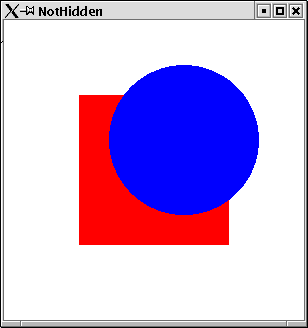Figure 6.1: Third dimension not correctly taken into account.
By default OpenGL does not take the depth into account. Shapes rendered later hide other shapes which were rendered earlier, neglecting the depth of the shapes. OpenGL provides a mechanism for automatically considering the depth of a shape. This simply needs to be activated. Three steps need to be done:
• as initial display mode WithDepthBuffer needs to be set.
• a depth function needs to be set. Usually the Less mode is used as function here. This ensures that closer objects hide objects farer away.
• the depth buffer needs to be cleared in the beginning of the display function.
Example:
Now we render the same to shapes as in the example before, but the depth machanism of OpenGL is activated.
Hidden
```import Graphics.Rendering.OpenGL
import Graphics.UI.GLUT  as GLUT

import Squares
import Circle
import PointsForRendering

main = do
(progName,_) <-  getArgsAndInitialize
initialDisplayMode \$= [WithDepthBuffer]

createWindow progName

depthFunc \$= Just Less
displayCallback \$= display
clearColor \$= Color4 1 1 1 1
mainLoop

display = do
clear [ColorBuffer,DepthBuffer]
translate (Vector3 0 0 (-0.5::GLfloat))
currentColor \$= Color4 1 0 0 1
square 1

translate (Vector3 0.2 0.2 (0.5::GLfloat))
currentColor \$= Color4 0 0 1 1
fillCircle 0.5
flush

```
Now as can be seen in figure 6.2, the red square hides parts of the blue circle.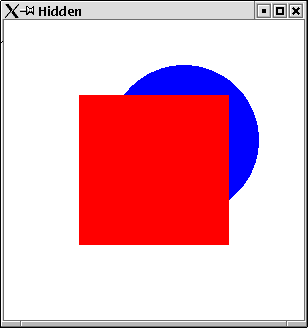Figure 6.2: Third dimension correctly taken into account by use of depth function.

## 6.2  Perspective Projection

In the real world objects closer to the viewer appear larger than objects farer away from the viewer. Up to now we only learnt how to set up an orthographic projection. In an orthographic projection objects farer away have the same size as object close to the viewer.
Example:
We can test the orthographic projection. Two squares equally in size, but in different distances from the viewer are rendered:
NotSmaller
```import Graphics.Rendering.OpenGL
import Graphics.UI.GLUT  as GLUT

import Squares
import Circle
import PointsForRendering

main = do
(progName,_) <-  getArgsAndInitialize
initialDisplayMode \$= [WithDepthBuffer]
createWindow progName
depthFunc \$= Just Less
displayCallback \$= display

matrixMode \$= Projection
ortho (-5) 5  (-5) 5 (1) 40
matrixMode \$= Modelview 0

clearColor \$= Color4 1 1 1 1
mainLoop

display = do
clear [ColorBuffer,DepthBuffer]
translate (Vector3 0 0 (-2::GLfloat))
currentColor \$= Color4 1 0 0 1
square 1

translate (Vector3 4 4 (-5::GLfloat))
currentColor \$= Color4 0 0 1 1
square 1
flush

```
As can be seen in figure 6.3 , the two squares have the same size, even though the red one is closer to the viewer.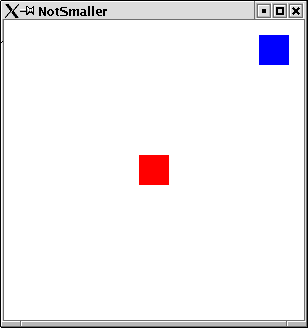Figure 6.3: Two squares in orthographic projection.
OpenGL provides the function frustum for specifying a perspective projection. frustum has 6 arguments:
• left: left bound for the closest orthogonal plane
• right: right bound for the closest orthogonal plane
• top: upper bound for the closest orthogonal plane
• bottom: lower bound for the closest orthogonal plane
• near: the closest things that can be seen
• far: the farest away things that can be seen
Figure 6.4 illustrates these six values.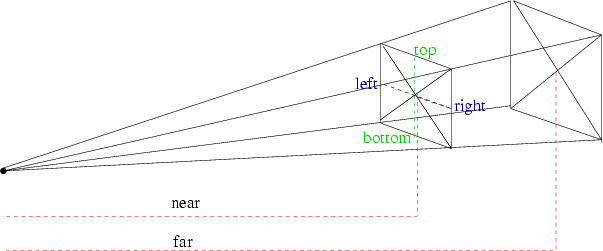Figure 6.4: Perspective projection with frustum.
Usually you will have negated values for top/botton and left/right.
Example:
Now we render the two squares from the previous example again. This time we use a perspective projection:
Smaller
```import Graphics.Rendering.OpenGL
import Graphics.UI.GLUT  as GLUT

import Squares
import Circle
import PointsForRendering

main = do
(progName,_) <-  getArgsAndInitialize
initialDisplayMode \$= [WithDepthBuffer]
createWindow progName
depthFunc \$= Just Less
displayCallback \$= display

matrixMode \$= Projection
let near   = 1
far    = 40
right  = 1
top    = 1
frustum (-right) right (-top) top near far
matrixMode \$= Modelview 0

clearColor \$= Color4 1 1 1 1
mainLoop

display = do
clear [ColorBuffer,DepthBuffer]
translate (Vector3 0 0 (-2::GLfloat))
currentColor \$= Color4 1 0 0 1
square 1

translate (Vector3 4 4 (-5::GLfloat))
currentColor \$= Color4 0 0 1 1
square 1
flush

```
Now, as can be seen in figure 6.5, the blue square appears to be smaller than the red square.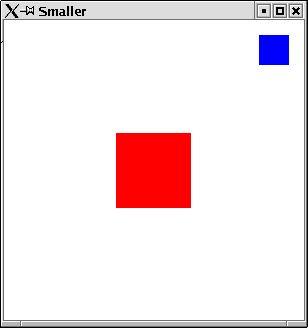Figure 6.5: Two squares in perspective projection.
HopenGL provides a second function to define a perspective projection: perspective. Here instead of left, right, top, bottom an angle between the top/bottom ray and the width of the closest plane can be specified.
Figure 6.6 illustrates these values.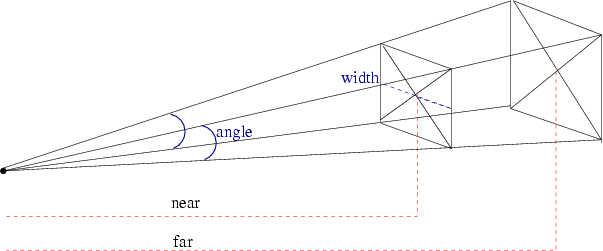Figure 6.6: Perspective projection.

## 6.3  Setting up the Point of View

In the previous section we have learnt that there is a second way how to project the three dimensional space onto the two dimensional area of the screen. We did however not yet specify, where in the three dimensional space the viewer is situated and in what direction they are looking. In order to define this, OpenGL provides the function lookAt. It has three arguments:
• the point, where the viewer is situated.
• the point at which the viewer is looking.
• and a vector, which specifies the direction which is to be up for the viewer.

### 6.3.1  Oribiting around the origin

The point of view, where we are looking from is interesting, when we change it. In the following a module is defined, which allows the viewer to move along a sphere. The point of view can be set for a given sphere position. The position is specified by two angles and a radius. The first angle defines which way to move around the x-axis the second angle, which angle to move around the y-axis. The radius defines the distance from the origin. The position can be changed through keyboard events.
OrbitPointOfView
```module OrbitPointOfView where

import Graphics.Rendering.OpenGL
import Graphics.UI.GLUT  as GLUT

import StateUtil
import Data.IORef

setPointOfView pPos = do
(alpha,beta,r) <- get pPos
let
(x,y,z)    = calculatePointOfView alpha beta r
(x2,y2,z2) = calculatePointOfView ((alpha+90)`mod` 360) beta r
lookAt (Vertex3 x y z) (Vertex3 0 0 0) (Vector3 x2 y2 z2)

calculatePointOfView  alp bet r =
let alpha =  fromIntegral alp*2*pi/fromIntegral 360
beta  =  fromIntegral bet*2*pi/fromIntegral 360
y = r * cos alpha
u = r * sin alpha
x = u * cos beta
z = u * sin beta
in (x,y,z)

keyForPos pPos (Char '+')         = modPos pPos (id,id,\x->x-0.1)
keyForPos pPos (Char '-')         = modPos pPos (id,id,(+)0.1)
keyForPos pPos (SpecialKey KeyLeft) = modPos pPos (id,(+)359,id)
keyForPos pPos (SpecialKey KeyRight)= modPos pPos (id,(+)1,id)
keyForPos pPos (SpecialKey KeyUp)   = modPos pPos ((+)1,id,id)
keyForPos pPos (SpecialKey KeyDown) = modPos pPos ((+)359,id,id)
keyForPos  _  _                     = return ()

modPos pPos (ffst,fsnd,ftrd) = do
(alpha,beta,r) <- get pPos
pPos \$= (ffst alpha `mod` 360,fsnd beta `mod` 360,ftrd r)
postRedisplay Nothing

reshape screenSize@(Size w h) = do
viewport \$= ((Position 0 0), screenSize)
matrixMode \$= Projection
let near   = 0.001
far    = 40
fov    = 90
ang    = (fov*pi)/(360)
top    = near / ( cos(ang) / sin(ang) )
aspect = fromIntegral(w)/fromIntegral(h)
right = top*aspect
frustum (-right) right (-top) top near far
matrixMode \$= Modelview 0

```
Example:
Let us use the module above, to orbit around a cube. Therefore we a define simple module, which renders a cube with differently colored areas. The cube is situated at the origin. We render the six areas by rendering a square at the origin and translate and rotate it into its final position.
ColorCube
```module ColorCube where

import Graphics.Rendering.OpenGL
import Graphics.UI.GLUT  as GLUT

import Squares
import StateUtil

locally = preservingMatrix

colorCube n = do
locally \$ do
currentColor \$= Color4 1 0 0 1
translate\$Vector3 0 0 (-n/2)
square n
locally \$ do
currentColor \$= Color4 0 1 0 1
translate\$Vector3 0 0 (n/2)
square n
locally \$ do
currentColor \$= Color4 0 0 1 1
translate\$Vector3 (n/2) 0 0
rotate 90 \$Vector3 0 (1::GLfloat) 0
square n
locally \$ do
currentColor \$= Color4 1 1 0 1
translate\$Vector3 (-n/2) 0 0
rotate 90 \$Vector3 0 (1::GLfloat) 0
square n
locally \$ do
currentColor \$= Color4 0 1 1 1
translate\$Vector3 0 (-n/2) 0
rotate 90 \$Vector3 (1::GLfloat) 0 0
square n
locally \$ do
currentColor \$= Color4 1 1 1 1
translate\$Vector3 0 (n/2) 0
rotate 90 \$Vector3 (1::GLfloat) 0 0
square n

```
The following program allows to use the cursor keys to move around a cube at the origin:
OrbitAroundCube
```import Graphics.Rendering.OpenGL
import Graphics.UI.GLUT  as GLUT

import Squares
import OrbitPointOfView
import StateUtil
import ColorCube

main = do
(progName,_) <-  getArgsAndInitialize
initialDisplayMode \$= [WithDepthBuffer,DoubleBuffered]
createWindow progName
depthFunc \$= Just Less

pPos <- new (90::Int,270::Int,2.0)
keyboardMouseCallback \$= Just (keyboard pPos)

displayCallback \$= display pPos
reshapeCallback \$= Just reshape
mainLoop

```
The display function sets the viewer's position before rendering the cube:
OrbitAroundCube
```display pPos = do
setPointOfView pPos
clear [ColorBuffer,DepthBuffer]
colorCube 1
swapBuffers

```
As keyboard function we map directly to the function defined in OrbitPointOfView.
OrbitAroundCube
```keyboard pPos c _  _ _ = keyForPos pPos c

```
An example how the colored cube can now be seen is given in figure 6.7.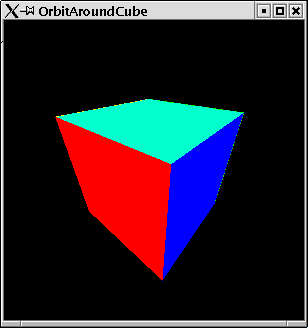Figure 6.7: A view of the colored cube,

## 6.4  3D Game: Rubik's Cube

In this section we implement a primitive version of Rubik's cube.
Rubik's cube in action can be found in figure 6.8.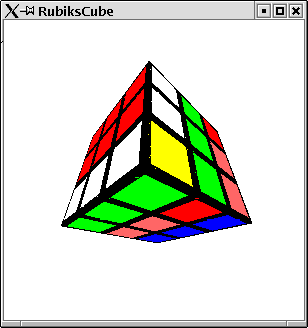Figure 6.8: Rubik's Cube in action.

### 6.4.1  Cube Logics

First of all we modell the logics of Rubic's Cube8.
A data type is provided for representation of a cube:
RubikLogic
```module RubikLogic where

data Rubik a
= Rubik (Front a) (Top a) (Back a) (Bottom a) (Left a) (Right a)

type Front a  = Area a
type Top a    = Area a
type Back a   = Area a
type Bottom a = Area a
type Left a   = Area a
type Right a  = Area a

type Area a = [Row a]
type Row a  = [a]

data AreaPosition =  Front |Top| Back| Bottom| Left| Right

data RubikColor = Red|Blue|Yellow|Green|Orange|White|Black

```
We make the type Rubik an instance of the class Functor:
RubikLogic
```instance Functor Rubik where
fmap f (Rubik front top back bottom left right)
= Rubik (mf front) (mf top) (mf back)
(mf bottom) (mf left) (mf right)
where
mf = map (map f)

```
The initial cube is defined
RubikLogic
```initCube  = Rubik (area Red)  (area Blue)  (area Yellow)
(area Green)(area Orange)(area White)

area c  = [[c,c,c],[c,c,c],[c,c,c]]

```
The main operation on a cube is to turn one of its six sides. The function rotateArea specifies, how this effects a cube.
RubikLogic
```rotateArea RubikLogic.Front
(Rubik front top back bottom left right) =
Rubik front' top' back bottom' left' right'
where
top'    = newRow 3 (reverse\$column 3 left) top
bottom' = newRow 1 (reverse\$column 1 right) bottom
left'   = newColumn 3 (row 1 bottom) left
right'  = newColumn 1 (row 3 top) right
front'  = rotateBy3 front

rotateArea RubikLogic.Back
(Rubik front top back bottom left right) =
Rubik front' top' back' bottom'
(rotateBy2 left') (rotateBy2 right')
where
(Rubik back' bottom' front' top' left' right') =
rotateArea RubikLogic.Front
(Rubik back bottom front top
(rotateBy2 left) (rotateBy2 right))

rotateArea RubikLogic.Bottom
(Rubik front top back bottom left right) =
Rubik front' top back' bottom' left' right'
where
back'   = newRow 1 (reverse\$row 3 left) back
front'  = newRow 3 (row 3 right) front
left'   = newRow 3 (row 3 front) left
right'  = newRow 3 (reverse\$row 1 back) right
bottom'  = rotateBy1 bottom

rotateArea RubikLogic.Top
(Rubik front top back bottom left right) =
Rubik front' top' back' bottom left' right'
where
back'   = newRow 3 (reverse\$row 1 right) back
front'  = newRow 1 (row 1 left) front
left'   = newRow 1 (reverse\$row 3 back) left
right'  = newRow 1 (row 1 front) right
top'  = rotateBy1 top

rotateArea RubikLogic.Left
(Rubik front top back bottom left right) =
Rubik front' top' back' bottom' left' right
where
top'    = newColumn 1 (column 1 front) top
bottom' = newColumn 1 (column 1 back) bottom
left'   = rotateBy3 left
back'   = newColumn 1 (column 1 top) back
front'  = newColumn 1 (column 1 bottom) front

rotateArea RubikLogic.Right
(Rubik front top back bottom left right) =
Rubik front' top' back' bottom' left right'
where
top'    = newColumn 3 (column 3 back) top
bottom' = newColumn 3 (column 3 front) bottom
right'  = rotateBy3 right
back'   = newColumn 3 (column 3 bottom) back
front'  = newColumn 3 (column 3 top) front

rotateBy1
[[x1,x2,x3]
,[x8,x,x4]
,[x7,x6,x5]] =
[[x3,x4,x5]
,[x2,x,x6]
,[x1,x8,x7]]

rotateBy2 =  rotateBy1 .rotateBy1
rotateBy3 =  rotateBy2 .rotateBy1

```
Finally some useful functions for manipulation of an area are given.
RubikLogic
```column n  = map (\row->row !! (n-1))

row n area = area !!(n-1)

newRow 1 row [a,r,ea] = [row,r,ea]
newRow 2 row [a,r,ea] = [a,row,ea]
newRow 3 row [a,r,ea] = [a,r,row]

newColumn n column area = map (doIt n) areaC
where
areaC =  zip area column
doIt 1 ((r:ow),c) = c:ow
doIt 2 ((r:o:w),c) = r:c:w
doIt 3 ((r:o:w:xs),c) = r:o:c:xs

```

### 6.4.2  Rendering the Cube

We have a logical modell of a cube. Now we can render this in a coordinate system. In an earlier section we allready provided a function to render one single side. We simply need to render the six sides and move them to the correct position.
RenderRubik
```module RenderRubik where

import Graphics.Rendering.OpenGL as OpenGL hiding (Red,Green,Blue)
import Graphics.UI.GLUT  as GLUT hiding (Red,Green,Blue)

import PointsForRendering
import RubikLogic
import RubikFace
import Squares

_FIELD_WIDTH :: GLfloat
_FIELD_WIDTH = 1/3

renderCube (Rubik front top back bottom left right) = do
render RubikLogic.Top     top
render RubikLogic.Back    back
render RubikLogic.Front   front
render RubikLogic.Bottom  bottom
render RubikLogic.Left    left
render RubikLogic.Right   right

render Top    cs = preservingMatrix\$do
translate \$Vector3 (1.5*_FIELD_WIDTH) 0 0
rotate (90)\$Vector3  0 1 (0::GLfloat)
renderCubeSide cs

render RubikLogic.Back   cs = preservingMatrix\$ do
translate \$Vector3 0 0 (-1.5*_FIELD_WIDTH)
rotate (180)\$Vector3 0 0 (1::GLfloat)
rotate (180)\$Vector3 1 0 (0::GLfloat)
renderCubeSide cs

render Bottom cs = preservingMatrix\$ do
translate \$Vector3  (-1.5*_FIELD_WIDTH) 0 0
rotate (270)\$Vector3  0 1 (0::GLfloat)
renderCubeSide cs

render RubikLogic.Front  cs = preservingMatrix\$ do
translate \$Vector3 0 0 (1.5*_FIELD_WIDTH)
renderCubeSide cs

render RubikLogic.Left   cs = preservingMatrix\$ do
translate \$Vector3 0 (1.5*_FIELD_WIDTH)  0
rotate (270) \$Vector3 1 0 (0::GLfloat)
renderCubeSide cs

render RubikLogic.Right  cs = preservingMatrix\$ do
translate \$Vector3 0 (-1.5*_FIELD_WIDTH)  0
rotate (270) \$Vector3 1 0 (0::GLfloat)
rotate (180)\$Vector3 1 0 (0::GLfloat)
renderCubeSide cs

renderCubeSide css = renderArea _FIELD_WIDTH  css

field = square _FIELD_WIDTH

```
The following function maps our abstract color type to concrete OpenGL colors:
RenderRubik
```doColor Red    = Color4 1 0 0 1.0
doColor Green  = Color4 0 1 0 1.0
doColor Blue   = Color4 0 0 1 1.0
doColor Yellow = Color4 1 1 0 1.0
doColor Orange = Color4 1 0.5 0.5 1
doColor White  = Color4 1 1 1 1.0
doColor Black  = Color4 0 0 0 1.0

```

### 6.4.3  Rubik's Cube

Finally we can create a simple application.
RubiksCube
```import Graphics.Rendering.OpenGL
import Graphics.UI.GLUT  as GLUT

import Data.IORef

import OrbitPointOfView
import StateUtil
import RubikLogic
import RenderRubik

main = do
initialDisplayMode \$= [DoubleBuffered,RGBMode,WithDepthBuffer]
(progName,_) <-  getArgsAndInitialize

createWindow progName

depthFunc \$= Just Less

pPos  <- new (90::Int,270::Int,2.0)
pCube <- new initCube

displayCallback \$= display pPos pCube
keyboardMouseCallback \$= Just (keyboard pPos pCube)

reshapeCallback \$= Just reshape
mainLoop

display pPos pCube = do
clearColor \$= Color4 1 1 1 1
clear [ColorBuffer,DepthBuffer]
setPointOfView pPos
cube <- get pCube
renderCube\$fmap doColor  cube
swapBuffers

keyboard _ pCube (Char '1') Down _ _
= rot pCube RubikLogic.Top
keyboard _ pCube (Char '2') Down _ _
= rot pCube RubikLogic.Bottom
keyboard _ pCube (Char '3') Down _ _
= rot pCube RubikLogic.Front
keyboard _ pCube (Char '4') Down _ _
= rot pCube RubikLogic.Back
keyboard _ pCube (Char '5') Down _ _
= rot pCube RubikLogic.Left
keyboard _ pCube (Char '6') Down _ _
= rot pCube RubikLogic.Right
keyboard pPos _   c         _    _ _
= keyForPos pPos c

rot pCube p = do
cube <- get pCube
pCube \$= rotateArea p cube
postRedisplay Nothing

```

## 6.5  Light

Let us begin with a simple 3-dimensional shape: a cube. A cube has six squares which we can render as the primitive shape Quad.
Cube
```module Cube where

import Graphics.Rendering.OpenGL
import Graphics.UI.GLUT  as GLUT

import PointsForRendering

cube l = renderAs Quads corners
where
corners =
[(l,0,l),(0,0,l),(0,l,l),(l,l,l)
,(l,l,l),(l,l,0),(l,0,0),(l,0,l)
,(0,0,0),(l,0,0),(l,0,l),(0,0,l)
,(l,l,0),(0,l,0),(0,0,0),(l,0,0)
,(0,l,l),(l,l,l),(l,l,0),(0,l,0)
,(0,l,l),(0,l,0),(0,0,0),(0,0,l)
]

```
Example:
We make a first try at rendering a cube:
RenderCube
```import Graphics.Rendering.OpenGL
import Graphics.UI.GLUT  as GLUT

import Cube

main = do
(progName,_) <-  getArgsAndInitialize
createWindow progName
displayCallback \$= display
mainLoop

display = do
clear [ColorBuffer]
rotate 40 (Vector3 1 1 (1::GLfloat))
cube 0.5
flush

```
The resulting window can be found in figure 6.9. It is not very exiting, we see a white shape, which has the outline of a cube, but do not get the three dimensional visual effect of a cube.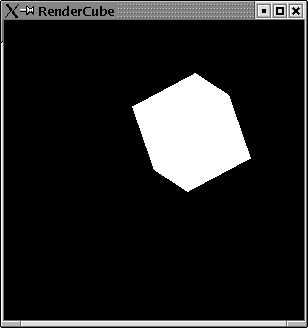Figure 6.9: An unlit cube.

### 6.5.1  Defining a light source

For rendering three dimensional objects it is not enough to specifiy their shapes and your viewing position. Crucial is the way the objects are illuminated. In order to get a three dimensional viesual effect on your two dimensional computer screen, it needs to be defined what kind of light source lights the object.
A light source can be specified fairly easy. First you need to set the state variable lighting to the value Enabled. Then you need to specify the position of your light source. This can be done by setting a special position state variable, e.g.  by
position (Light 0) \$= Vertex4 0.8 0 3.0 5.0.
And finally you need to turn the light source on by setting its state variable to enabled: light (Light 0) \$= Enabled.
Example:
Now we can render a cube with a defined light source:
LightCube
```import Graphics.Rendering.OpenGL
import Graphics.UI.GLUT  as GLUT

import Cube

main = do
(progName,_) <-  getArgsAndInitialize

depthFunc \$= Just Less

createWindow progName

lighting \$= Enabled
position (Light 0) \$= Vertex4 1 0.4 0.8  1
light (Light 0) \$= Enabled

displayCallback \$= display
mainLoop

display = do
clear [ColorBuffer]
rotate 40 (Vector3 1 1 (1::GLfloat))
cube 0.5
flush

```
The resulting window can be found in figure 6.10. Now we can identify a bit more the cube.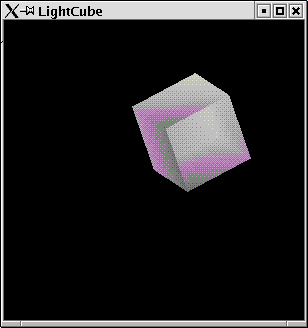Figure 6.10: A lit cube.
You might wonder, why the vertex for the light source position has four parameters. The forth parameter is a value by which the other three (the x, y, z coordinates) get divided.

### 6.5.2  Tux the Penguin

Let us render some cute object: Tux the penguin. We will roughly use the data from the OpenGL game tuxracer. The nice thing about a penguin is, that you can built it almost completely out of spheres. We will render Tux simply by rendering spheres, which are scaled to different forms and moved to the correct position.

#### Overall Setup

Tux
```import Graphics.Rendering.OpenGL as OpenGL
import Graphics.UI.GLUT  as GLUT

import OrbitPointOfView
import StateUtil

main = do
(progName,_) <-  getArgsAndInitialize
initialDisplayMode \$= [WithDepthBuffer,DoubleBuffered]
pPos <- new (90::Int,270::Int,1.0)
depthFunc \$= Just Less
createWindow progName

lighting  \$= Enabled
normalize \$= Enabled
depthFunc \$= Just Less

position (Light 0) \$= Vertex4 0 0 (10) 0
ambient (Light 0) \$= Color4 1 1 1 1
diffuse (Light 0) \$= Color4 1 1 1 1
specular (Light 0) \$= Color4 1 1 1 1
light (Light 0) \$= Enabled

displayCallback \$= display pPos
keyboardMouseCallback \$= Just (keyboard pPos)
reshapeCallback \$= Just reshape
mainLoop

keyboard pPos c _  _ _ = keyForPos pPos c

```
The main display function clears the necessary buffers and calls the main function for rendering the penguin Tux.
Tux
```display pPos = do
clearColor \$= Color4 1 0 0 1
setPointOfView pPos
clear [ColorBuffer,DepthBuffer]
tux
swapBuffers

```

#### Auxilliary Functions

We will use some auxilliary functions. First of all a function, which renders a scaled sphere.
Tux
```sphere r xs ys zs = do
scal xs ys zs
createSphere r

createSphere r = renderObject Solid \$Sphere' r 50 50

scal:: GLfloat -> GLfloat -> GLfloat -> IO ()
scal x y z = scale x y z

```
Furthermore some functions for easy translate and rotate transformations:
Tux
```transl:: GLfloat -> GLfloat -> GLfloat -> IO ()
transl x y z= translate\$Vector3 x y z

rota:: GLfloat -> GLfloat -> GLfloat -> GLfloat -> IO ()
rota a x y z  = rotate a \$ Vector3 x y z

rotateZ a = rota a 0 0 1
rotateY a = rota a 0 1 0
rotateX a = rota a 1 0 0

```
And eventually some functions to set the material properties for the different parts of a penguin.
Tux
```crMat (rd,gd,bd) (rs,gs,bs) exp = do
materialDiffuse   Front \$= Color4 rd gd bd  1.0
materialAmbient   Front \$= Color4 rd gd bd  1.0
materialSpecular  Front \$= Color4 rs gs bs  1.0
materialShininess Front \$= exp

materialDiffuse   Back \$= Color4 rd gd bd  1.0
materialSpecular  Back \$= Color4 rs gs bs  1.0
materialShininess Back \$= exp

whitePenguin = crMat (0.58, 0.58, 0.58)(0.2, 0.2, 0.2) 50.0
blackPenguin = crMat (0.1, 0.1, 0.1)   (0.5, 0.5, 0.5) 20.0
beakColour   = crMat (0.64, 0.54, 0.06)(0.4, 0.4, 0.4) 5
nostrilColour= crMat (0.48039, 0.318627, 0.033725)(0.0,0.0,0.0) 1
irisColour   = crMat (0.01, 0.01, 0.01)(0.4, 0.4, 0.4) 90.0

```

The neck and torso of a penguin are almost black spheres with some white front parts. We will modell such figures by setting s white sphere in front of a black sphere.
Tux
```makeBody = do
preservingMatrix\$do
blackPenguin
sphere 1 0.95 1.0 0.8
preservingMatrix\$do
whitePenguin
transl 0 0 0.17
sphere 1 0.8 0.9 0.7

```
The resulting image can be found in figure 6.11.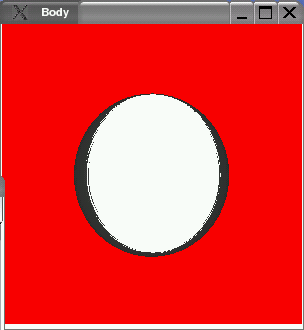Figure 6.11: Basic part for a penguin torso.
Torso and shoulders are scaled body parts:
Tux
```createTorso = preservingMatrix\$do
scal 0.9 0.9 0.9
makeBody

createShoulders = preservingMatrix\$do
transl 0 0.4 0.05
leftArm
rightArm
scal 0.72 0.72 0.72
makeBody

```
The resulting image for torso and shoulders can be found in figure 6.12.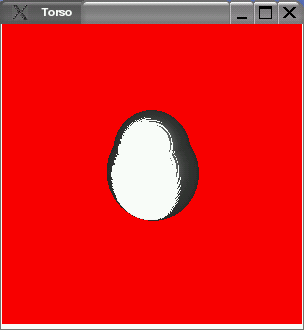Figure 6.12: Penguin torso and shoulders.
Tux
```createNeck = preservingMatrix\$do
transl 0 0.9 0.07
rotateY 90
blackPenguin
sphere 0.8 0.45 0.5 0.45
transl 0 (-0.08) 0.35
whitePenguin
sphere 0.66 0.8 0.9 0.7

transl 0 0.3 0.07
createBeak
createEyes
rotateY 90
blackPenguin
sphere 1 0.42 0.5 0.42

createBeak = do
preservingMatrix\$do
transl 0 (-0.205) 0.3
rotateX 10
beakColour
sphere 0.8 0.23 0.12 0.4
preservingMatrix\$do
beakColour
transl 0 (-0.23) 0.3
rotateX 10
sphere 0.66 0.21 0.17 0.38

```

#### Eyes

Tux
```createEyes = preservingMatrix\$do
leftEye
leftIris
rightEye
rightIris

leftEye = preservingMatrix\$do
transl 0.13 (-0.03) 0.38
rotateY 18
rotateZ 5
rotateX 5
whitePenguin
sphere 0.66 0.1 0.13 0.03

rightEye = preservingMatrix\$do
transl (-0.13) (-0.03) 0.38
rotateY (-18)
rotateZ (-5)
rotateX 5
whitePenguin
sphere 0.66 0.1 0.13 0.03

leftIris = preservingMatrix\$do
transl 0.12 (-0.045) 0.4
rotateY 18
rotateZ 5
rotateX 5
irisColour
sphere 0.66 0.055 0.07 0.03

rightIris = preservingMatrix\$do
transl (-0.12) (-0.045) 0.4
rotateY (-18)
rotateZ (-5)
rotateX 5
irisColour
sphere 0.66 0.055 0.07 0.03

```

#### Legs

Tux
```leftArm = preservingMatrix\$do
rotateY 180
transl (-0.56) 0.3 0
rotateZ 45
rotateX 90
leftForeArm
blackPenguin
sphere 0.66 0.34 0.1 0.2

rightArm = preservingMatrix\$do
transl (-0.56) 0.3 0
rotateZ 45
rotateX(-90)
rightForeArm
blackPenguin
sphere 0.66 0.34 0.1 0.2

leftForeArm = preservingMatrix\$do
transl (-0.23) 0 0
rotateZ 20
rotateX 90
leftHand
blackPenguin
sphere 0.66 0.3 0.07 0.15

rightForeArm = leftForeArm

leftHand = preservingMatrix\$do
transl (-0.24) 0 0
rotateZ 20
rotateX 90
blackPenguin
sphere 0.5 0.12 0.05 0.12

leftTigh = preservingMatrix\$do
rotateY 180
transl (-0.28) (-0.8) 0
rotateY 110
leftHipBall
leftCalf

rotateY (-110)
transl 0 (-0.1) 0
beakColour
sphere 0.5 0.07 0.3 0.07

leftHipBall = preservingMatrix\$do
blackPenguin
sphere 0.5 0.09 0.18 0.09

rightTigh = preservingMatrix\$do
transl (-0.28) (-0.8) 0
rotateY (-110)
rightHipBall
rightCalf

transl 0 (-0.1) 0
beakColour
sphere 0.5 0.07 0.3 0.07

rightHipBall = preservingMatrix\$do
blackPenguin
sphere 0.5 0.09 0.18 0.09

leftCalf = preservingMatrix\$do
transl 0 (-0.21) 0
rotateY 90
leftFoot
beakColour
sphere 0.5 0.06 0.18 0.06

rightCalf = preservingMatrix\$do
transl 0 (-0.21) 0
rightFoot
beakColour
sphere 0.5 0.06 0.18 0.06

```

#### Feet

Tux
```foot = preservingMatrix\$do
scal  1.1 1.0 1.3
beakColour
footBase
toe1
toe2
toe3

footBase = preservingMatrix\$do
sphere 0.66 0.25 0.08 0.18

toe1 = preservingMatrix\$do
transl (-0.07) 0 0.1
rotateY 30
scal 0.27 0.07 0.11
createSphere 0.66

toe2 = preservingMatrix\$do
transl (-0.07) 0 (-0.1)
rotateY (-30)
sphere 0.66  0.27 0.07 0.11

toe3 = preservingMatrix\$do
transl (-0.08) 0 0
sphere 0.66  0.27 0.07 0.10

leftFoot = preservingMatrix\$do
transl 0 (-0.09) 0
rotateY (100)
foot

rightFoot = preservingMatrix\$do
transl 0 (-0.09) 0
rotateY 180
foot

```
The resulting image can be found in figure 6.13.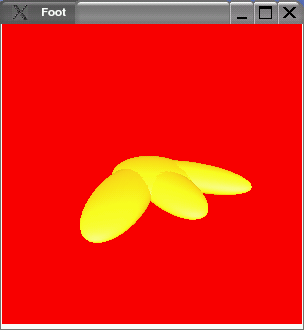Figure 6.13: A penguin foot.

#### Tail

Tux
```createTail = preservingMatrix\$ do
transl 0 (-0.4) (-0.5)
rotateX (-60)
transl 0 0.15 0
blackPenguin
sphere 0.5 0.2 0.3 0.1

```

#### The complete penguin

We can use all the parts to define Tux.
Tux
```tux = preservingMatrix\$do
scale 0.35 0.35 (0.35::GLfloat)
rotateY (-180)
rotateZ (-180)
createTorso
createShoulders
createNeck
leftTigh
rightTigh
createTail

```
The resulting window can be found in figure 6.14.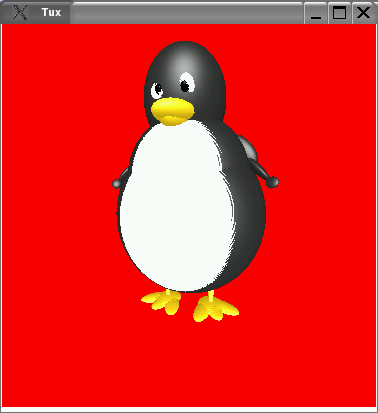Figure 6.14: Tux the penguin..

## Bibliography

[]
Norman Chin, Chris Frazier, Paul Ho, Zicheng Lui, and Kevin P. Smith. The OpenGL Graphics System Utility Library, 1998. ftp://ftp.sgi.com/opengl/doc/opengl1.2/glu1.3.ps.
[]
Mark J. Kilgard. The OpenGL Utility Toolkit (GLUT), 1996. www.opengl.org/developers/documentation/glut/glut-3.spec.ps.
[]
Simon L. Peyton Jones and Philip Wadler. Imperative functional programming. In Proceedings 20th Symposium on Principles of Programming Languages, pages 71-84, Charleston, South Carolina, 1993. ACM.
[]
P. Wadler. Comprehending monads. In Proceedings of Symposium on Lisp and Functional Programming, pages 61-78, Nice, France, June 1990. ACM.
[]
Mason Woo, OpenGL Architecture Review Board, Jackie Neider, Tom Davis, and Dave Shreiner. OpenGL Programming Guide 3rd Edition. Addison-Wesley, Reading, MA, 1997.

### Footnotes:

1Similar name different person.
2Variables bound in let-expressions are not variables as known from imperative languages. Line 4 in the example above does not assign a new value to a variable x but defines a new variable x.
3Unlike the object orientated languages Java, which misses an easy way to pass functions around.
4A nicer choice for this operator would have been :=, but this is not allowed for a function operator in Haskell, but denotes an infix constructor.
5Otherwise you might see arbitrary parts of other applications in your window frame.
6Which somehow does not look like the expected?
7This is no longer necessary, since this has been integrated into the HOpenGL library.
8There are certainly cleverer ways to do this, but I did not take the time to think of them.

File translated from TEX by TTH, version 3.20.
On 24 Sep 2004, 13:20.